# RD Sharma Solutions for Class 10 Maths Chapter 16 Surface Areas and Volumes

## RD Sharma Solutions Class 10 Maths Chapter 16 – Download Free PDF Updated for 2022-2023

RD Sharma Solutions for Class 10 Maths Chapter 16 – Surface Areas and Volumes are provided here to enhance knowledge of the covered concepts and score good marks in the exams. The Applications of Mathematics in everyday life are enormous. One such application is to find the surface areas and volumes of different solids and their combinations. This chapter is one of the important chapters in Class 10 Mathematics. Experienced teachers developed the RD Sharma Solutions for students to strengthen conceptual knowledge and solve problems with competence.

Chapter 16 – Surface Areas and Volumes contains three exercises. Students can find precise step-wise answers to these exercise problems in RD Sharma Solutions for Class 10 Maths. Subject-matter experts have developed these solutions to match the standards of all levels of students. In this chapter, students will be solving problems on finding surface areas and volumes of combinations of solids like a cuboid, cube, right circular cylinder, right circular cone, sphere, hemisphere and frustum of a cone.

## Download the PDF of RD Sharma Solutions for Class 10 Maths Chapter 16 Surface Areas and Volumes Here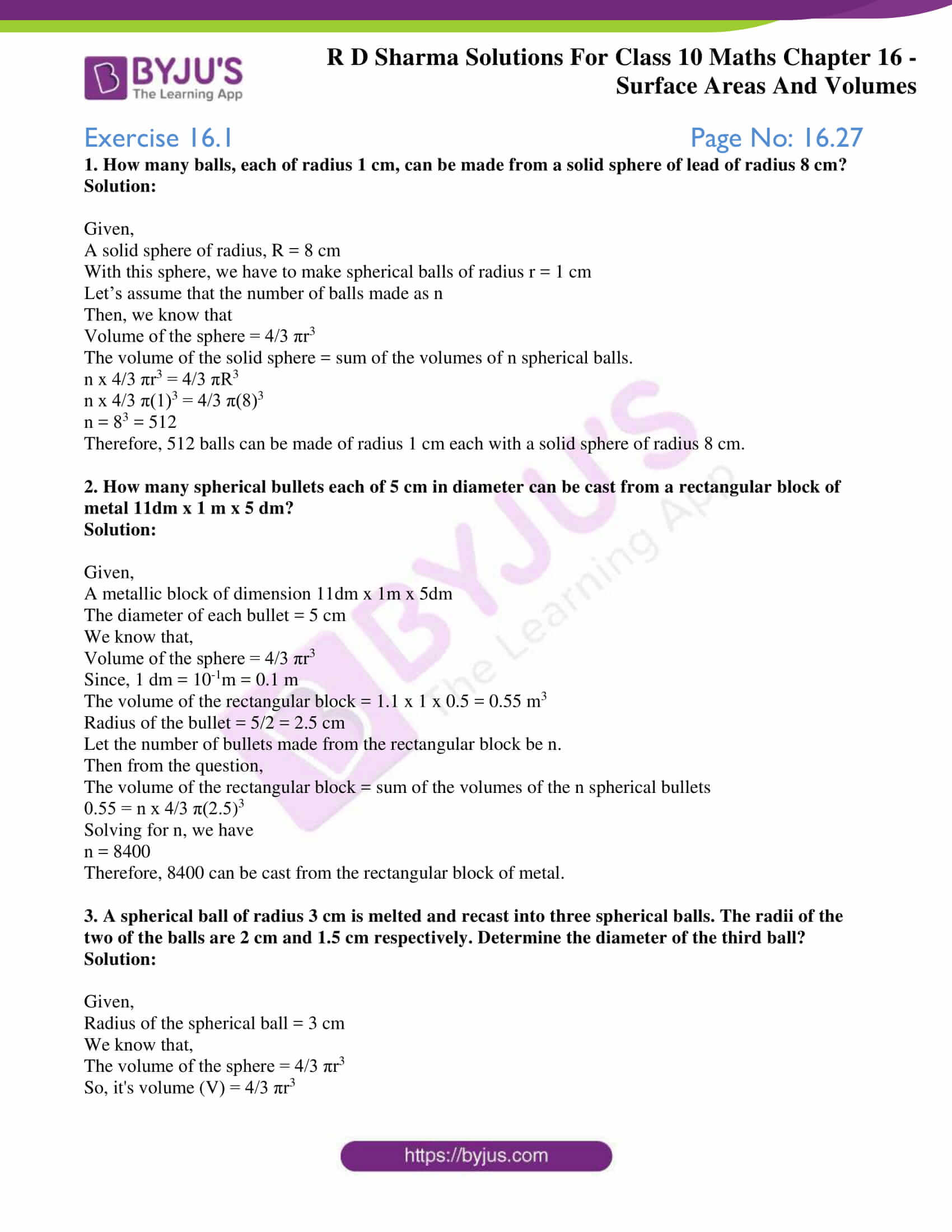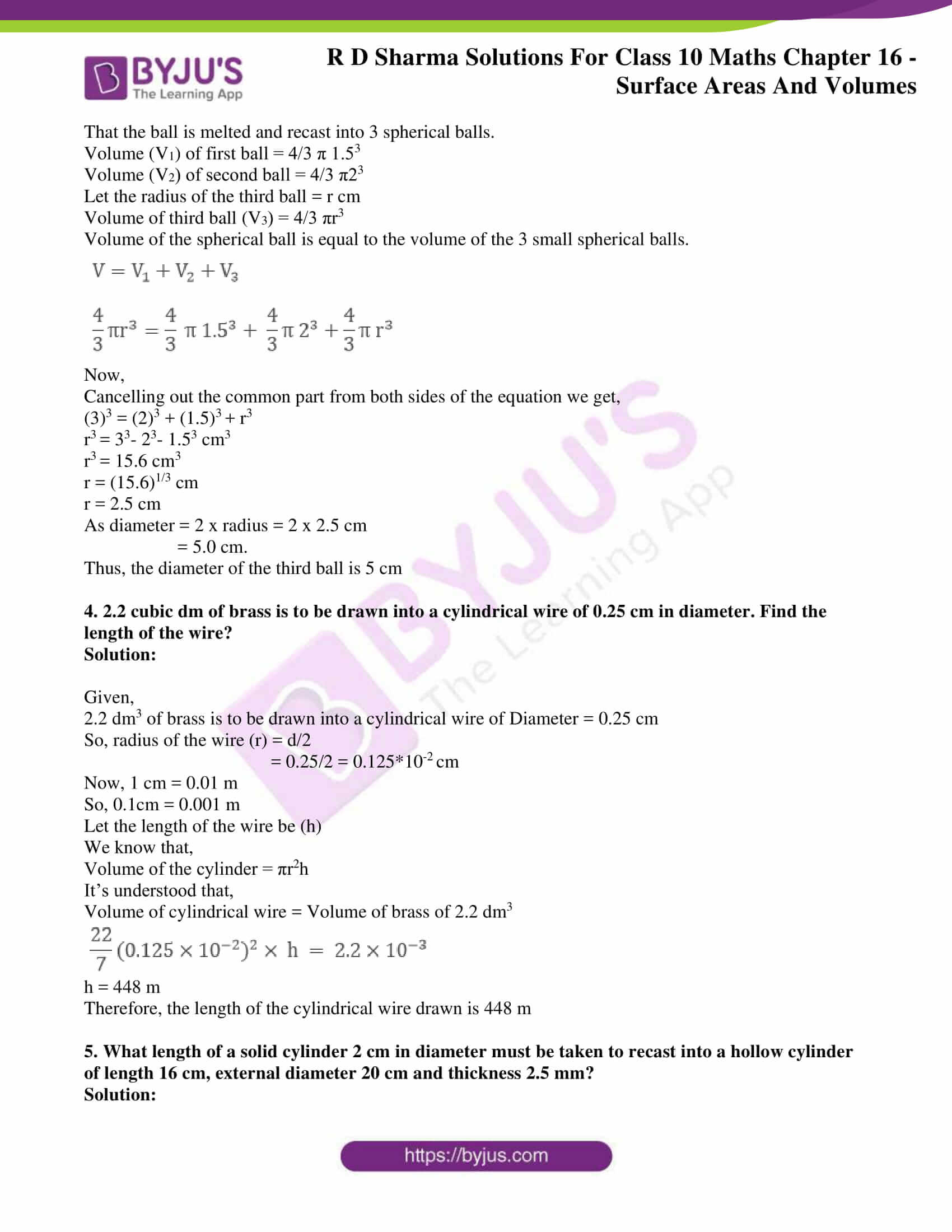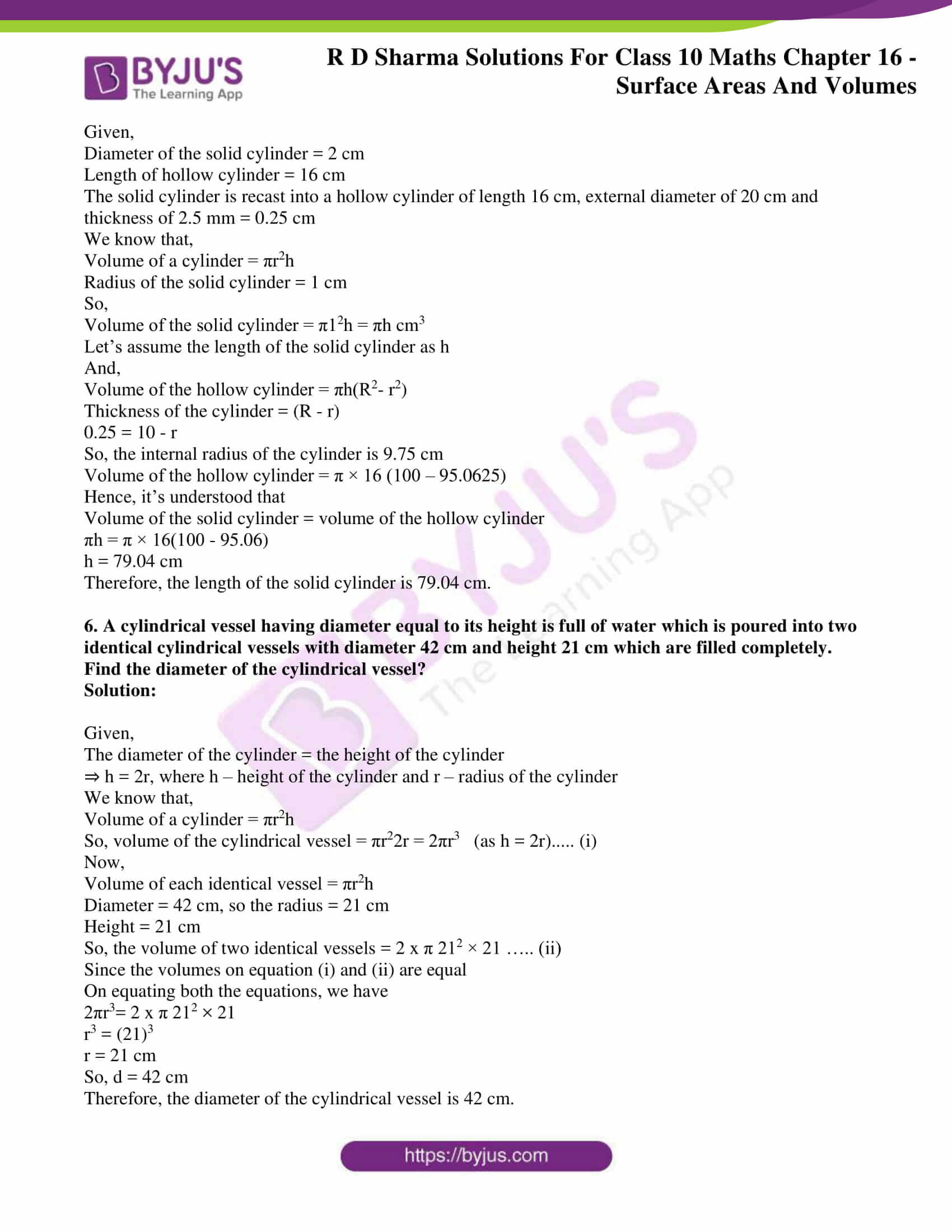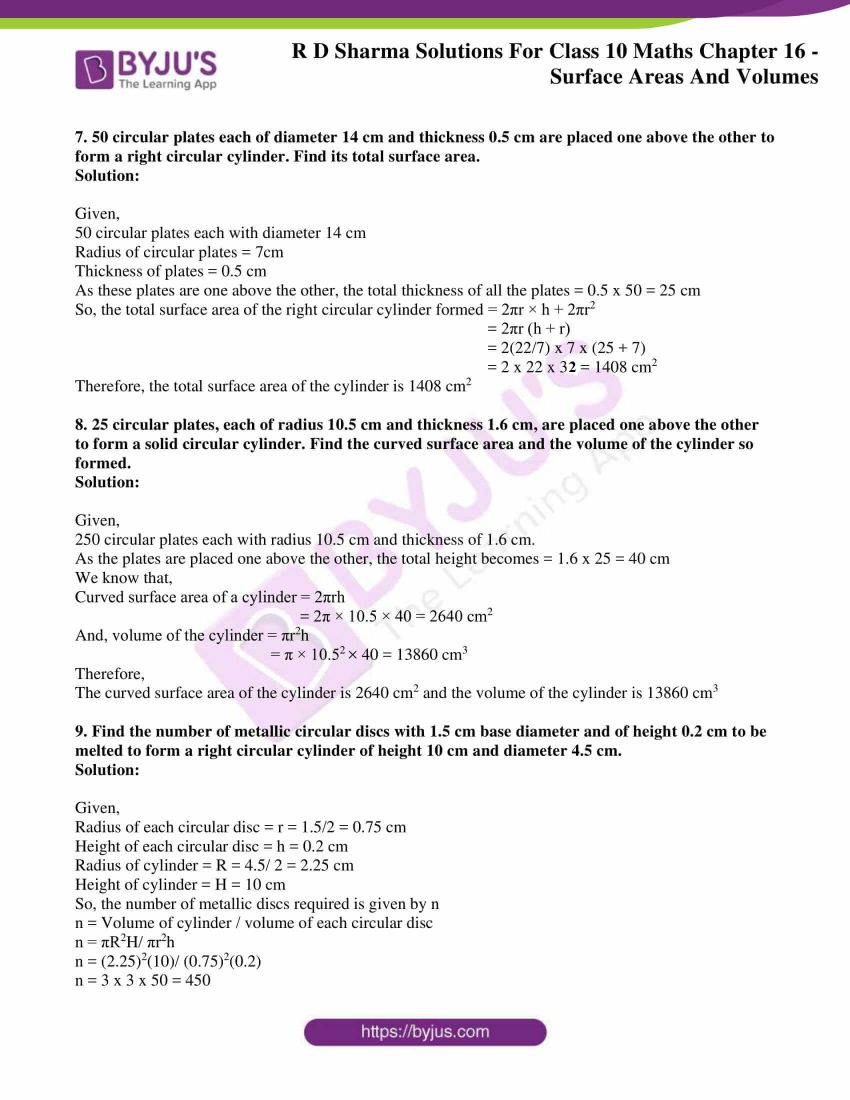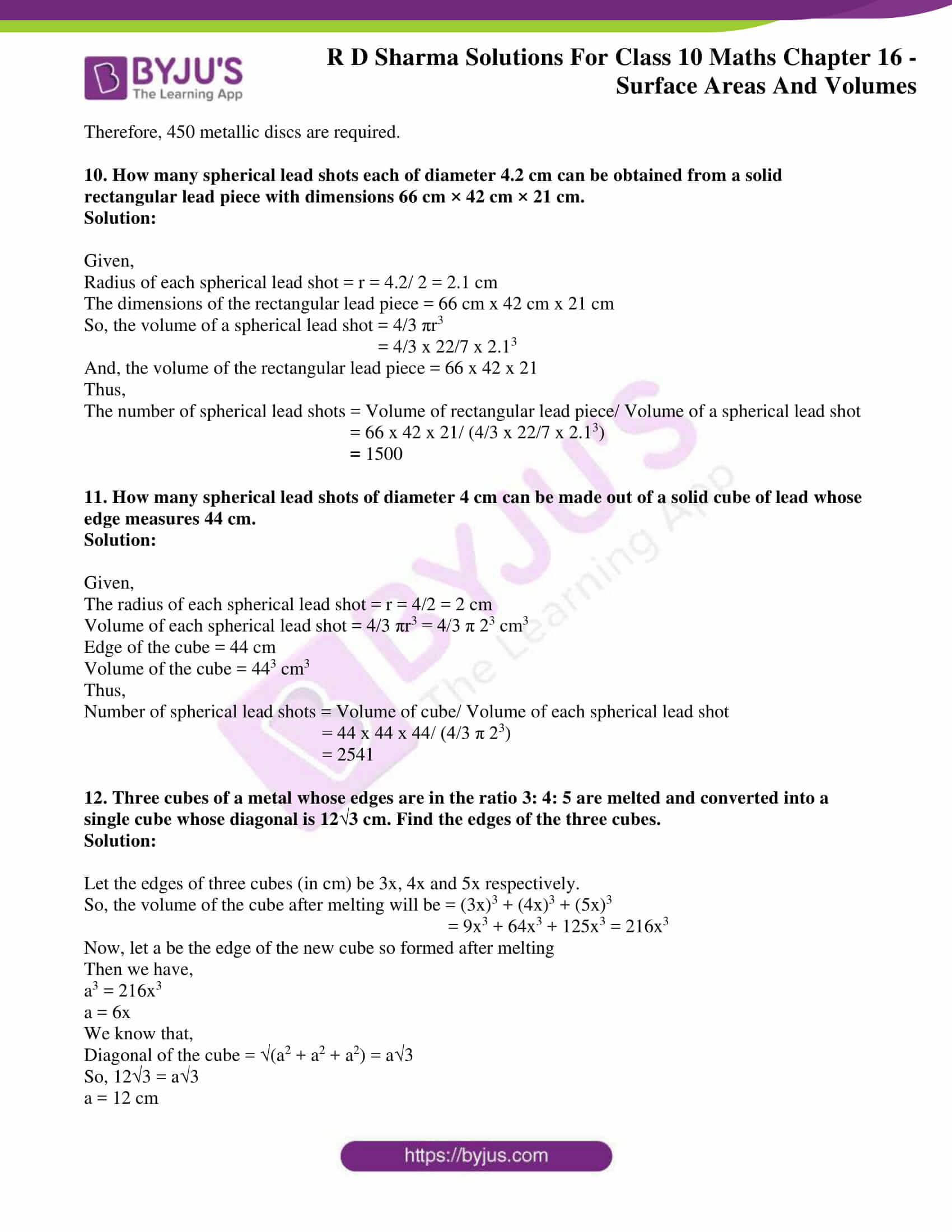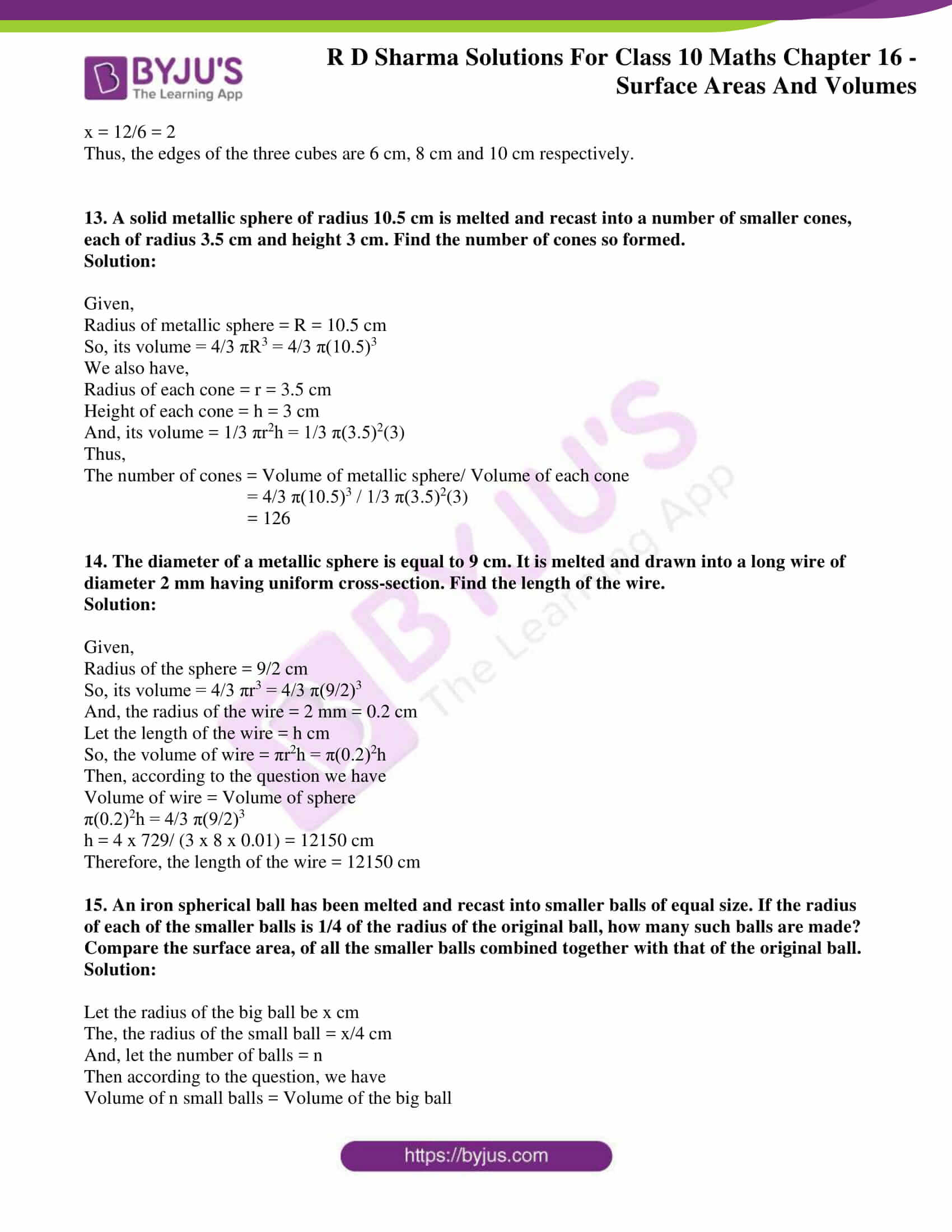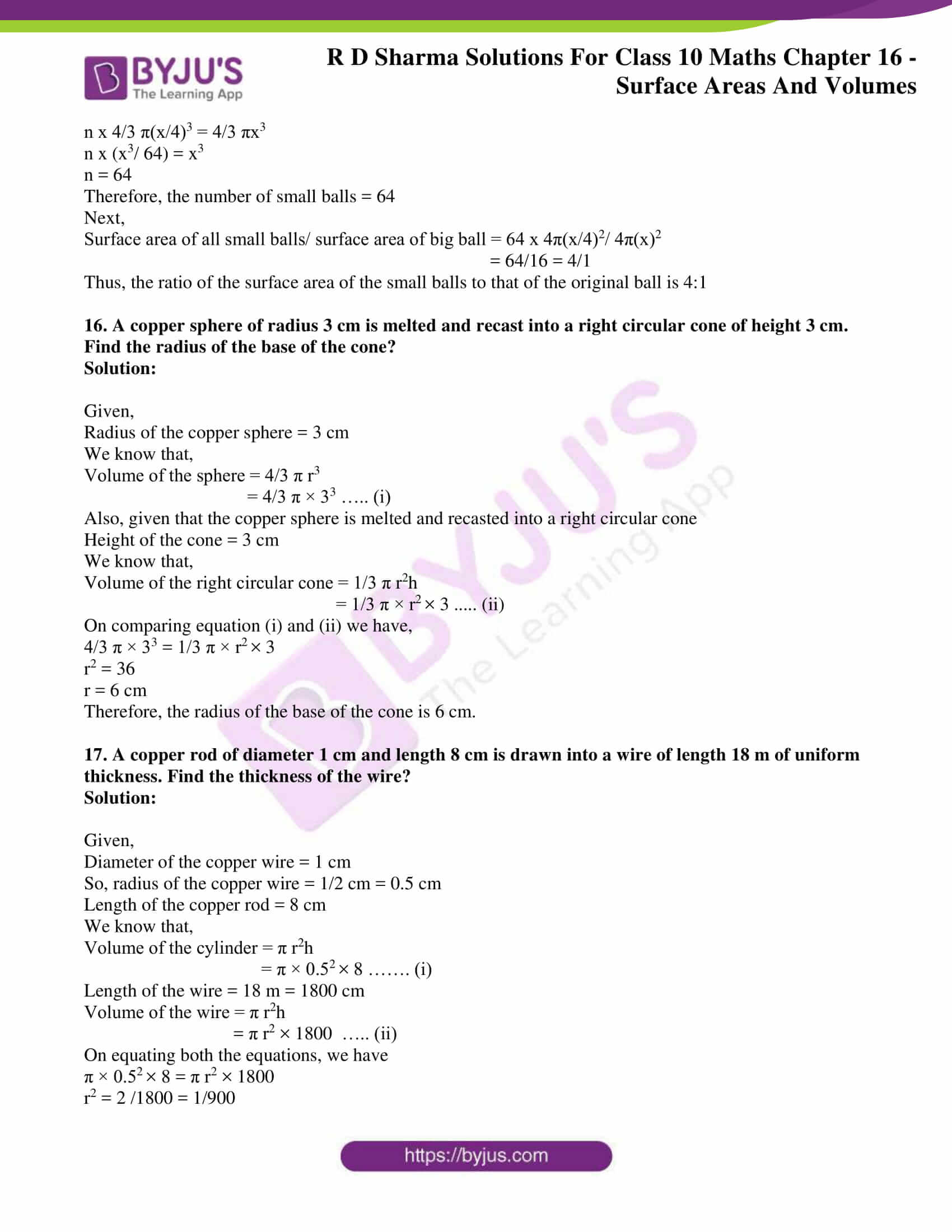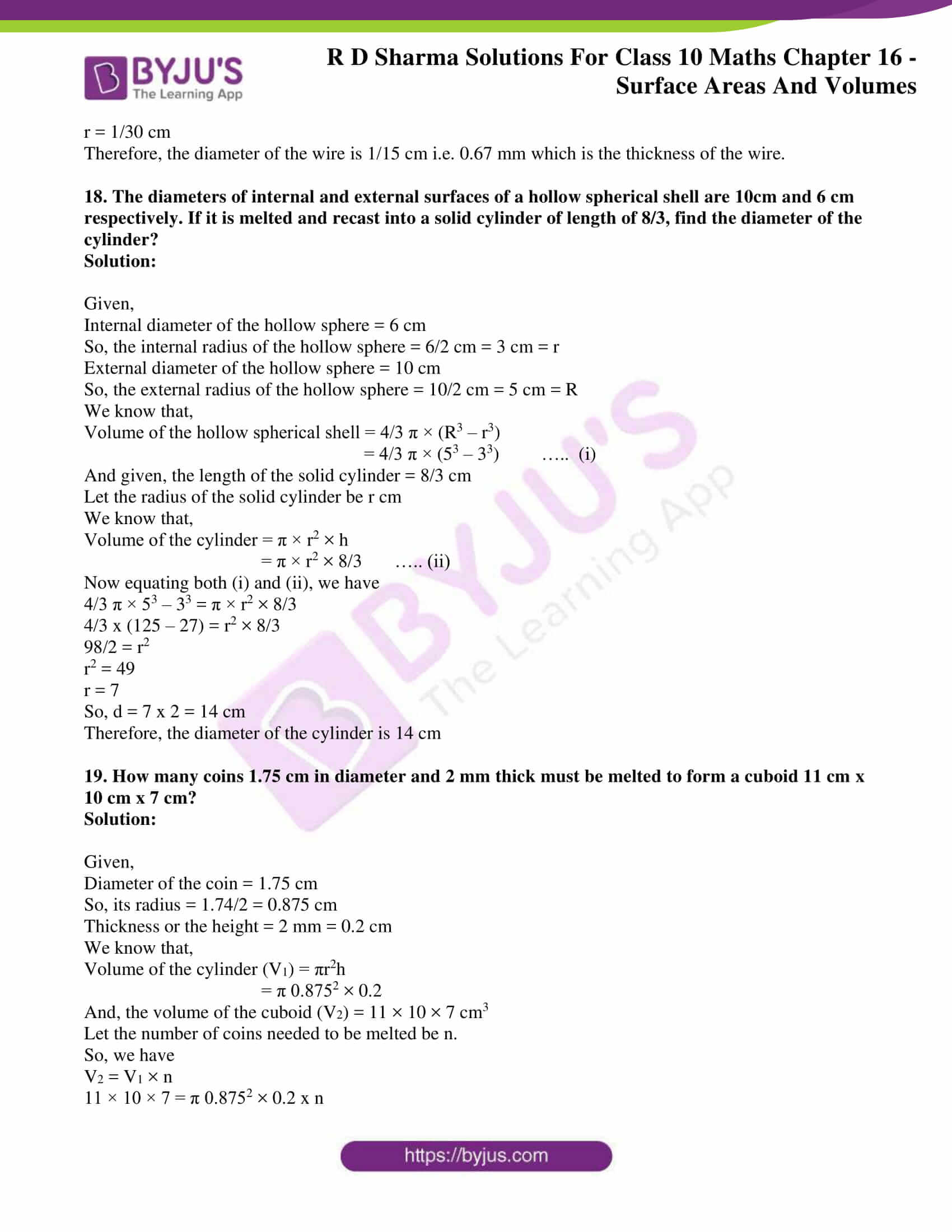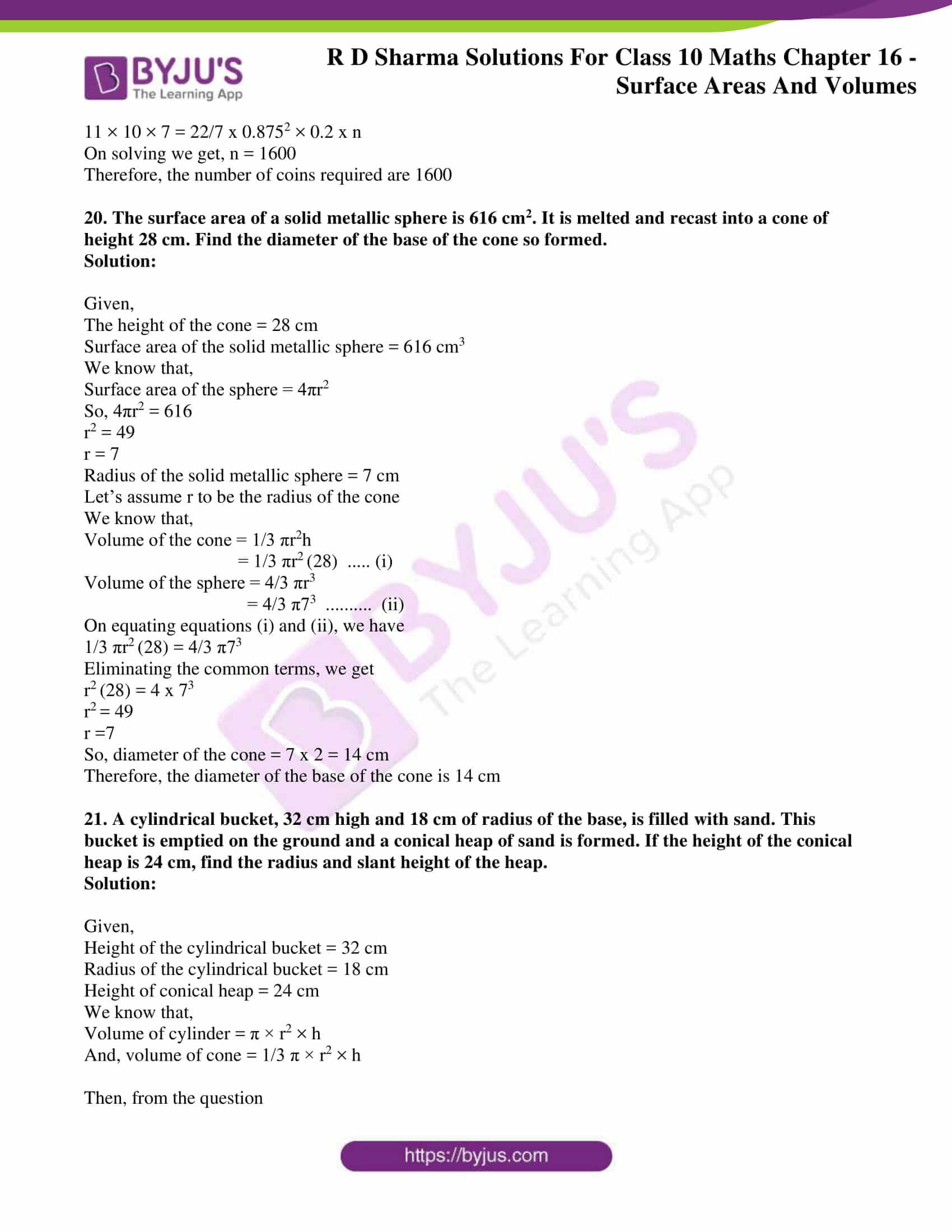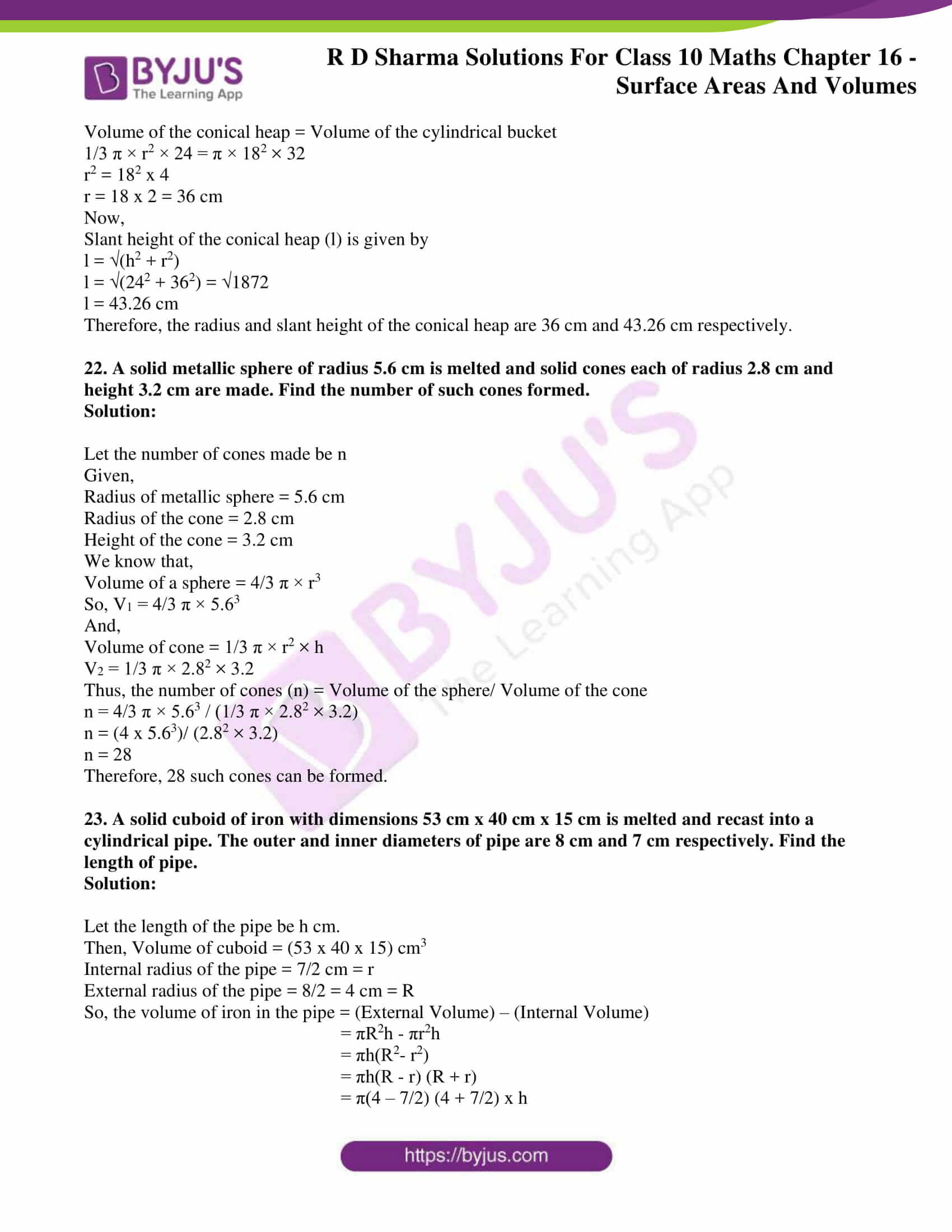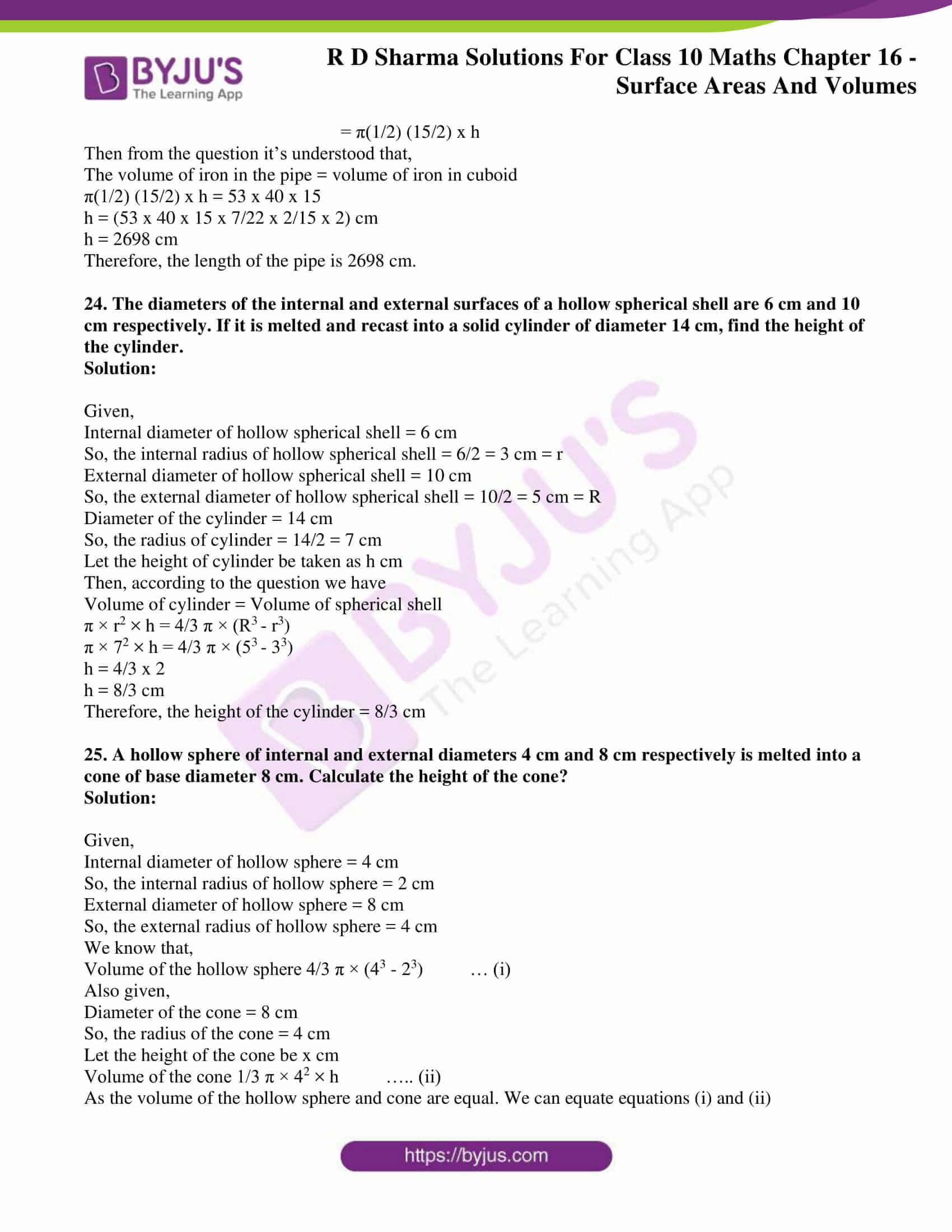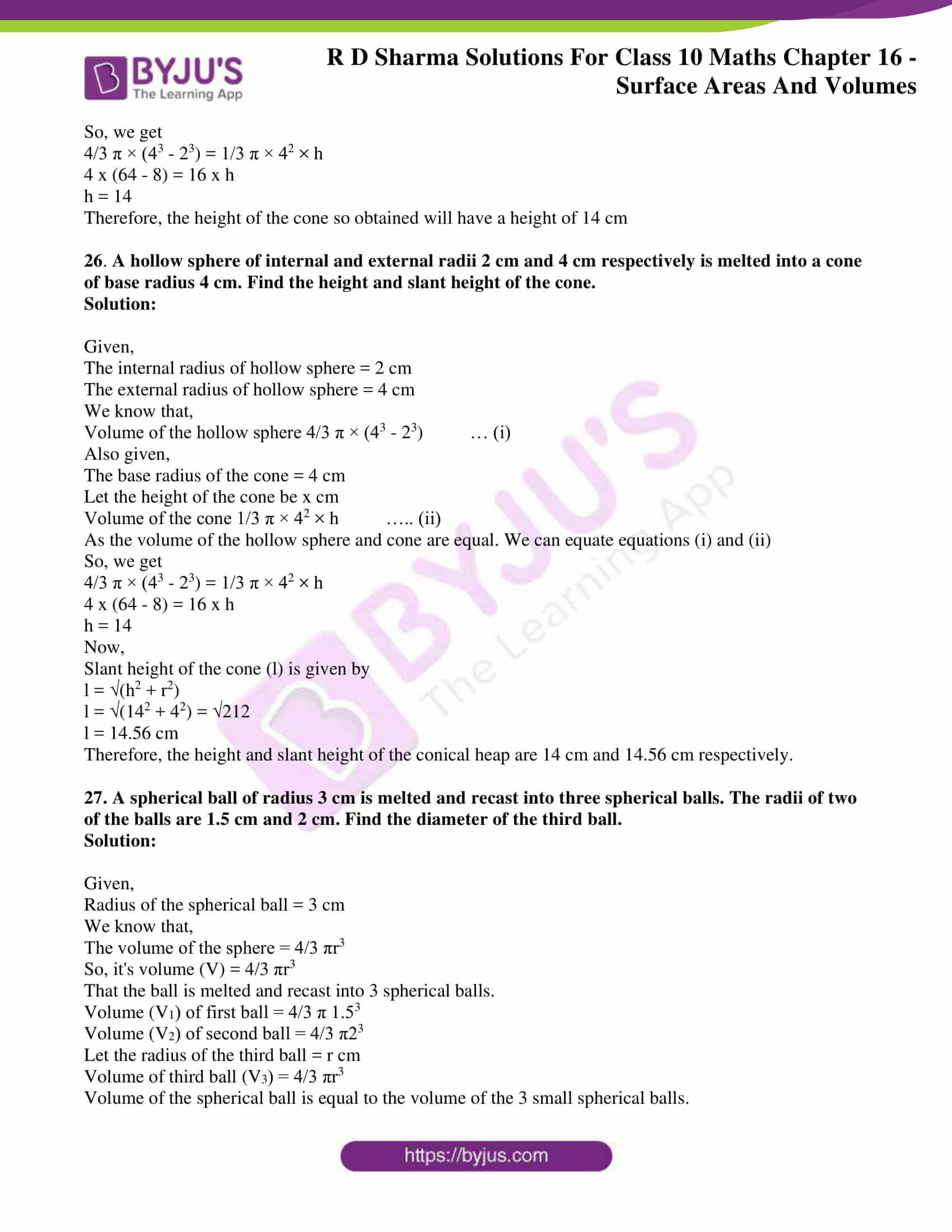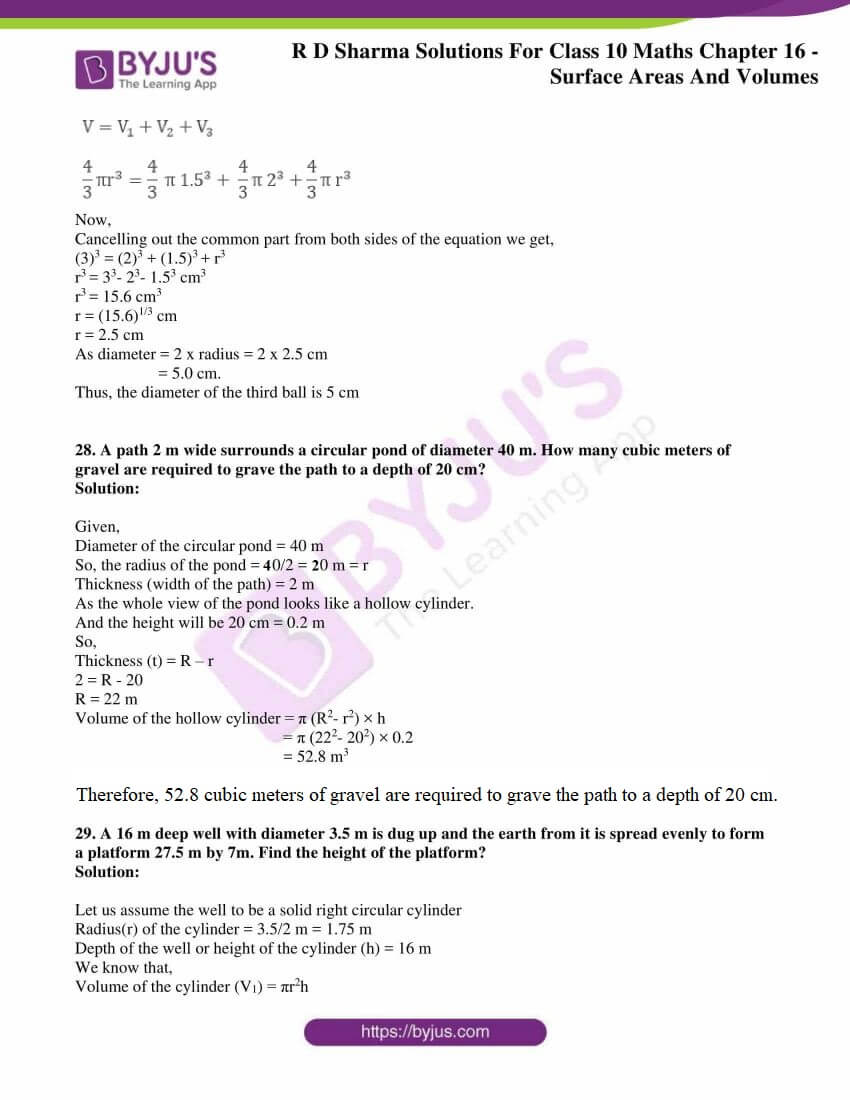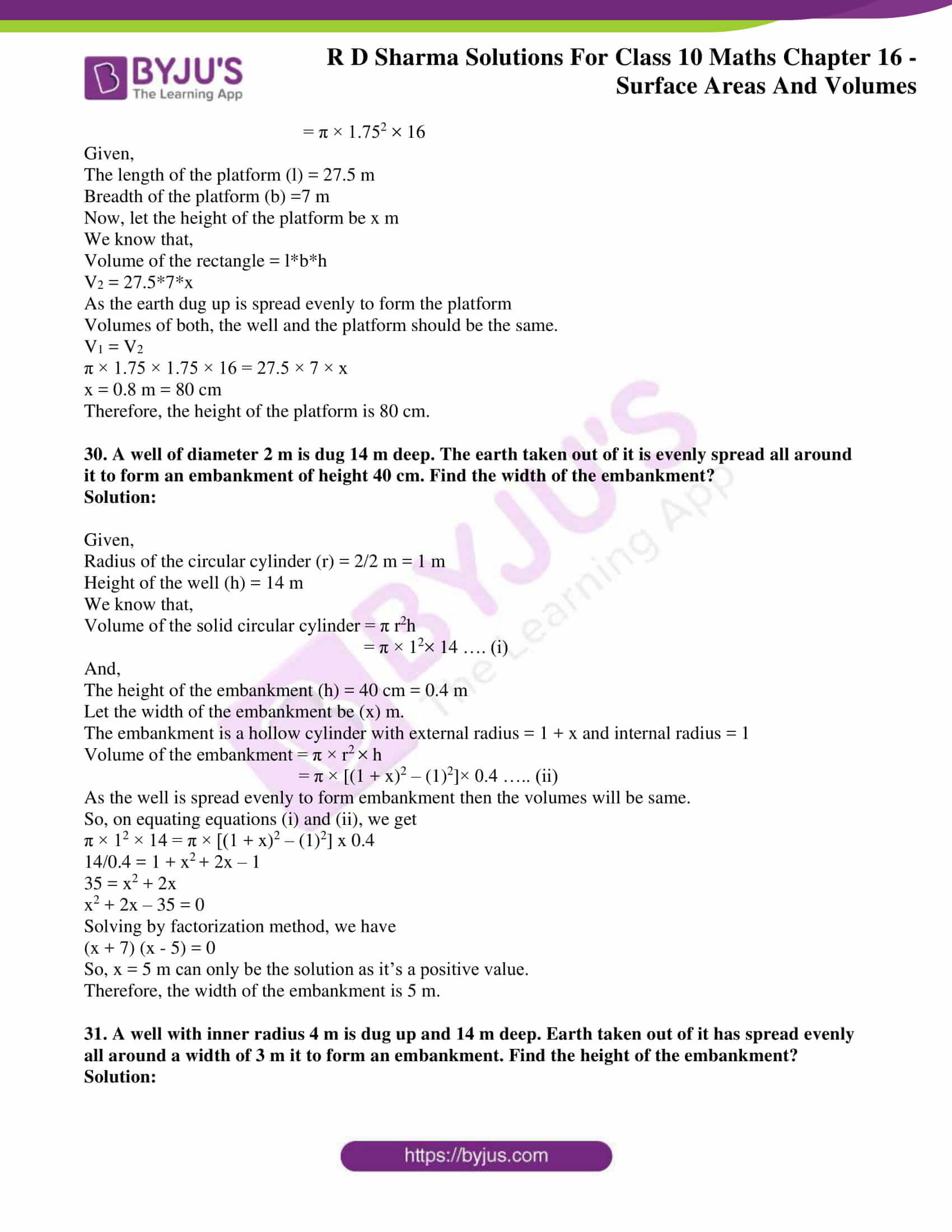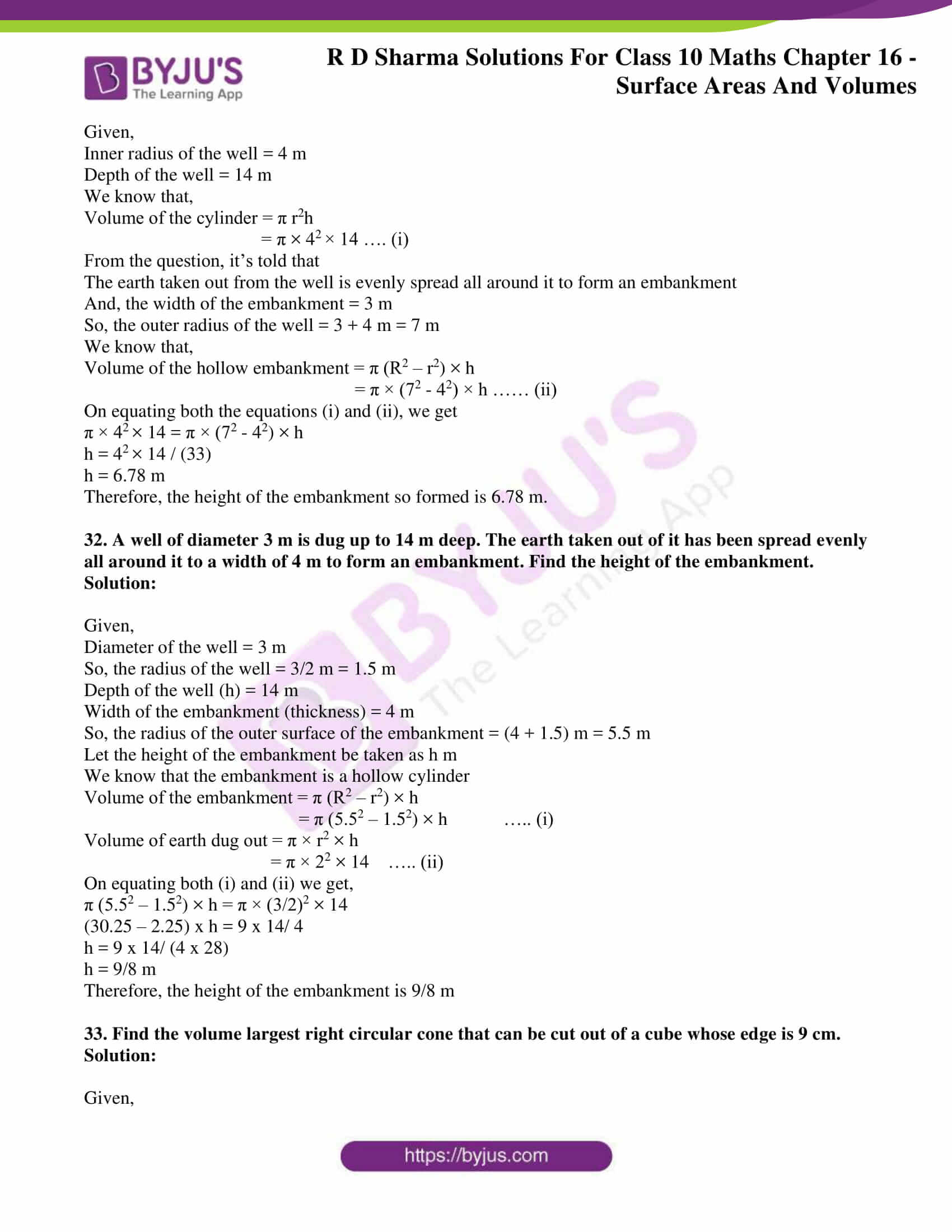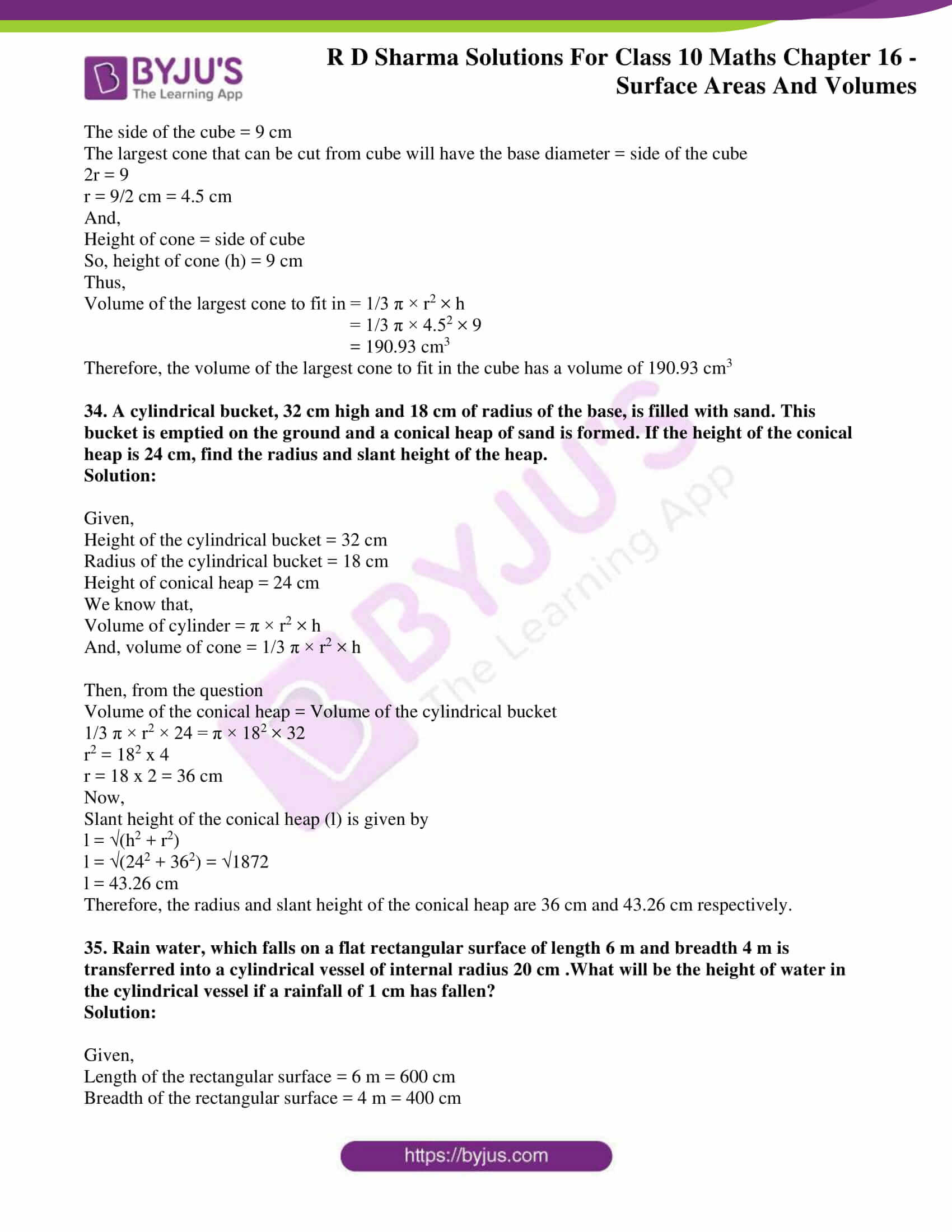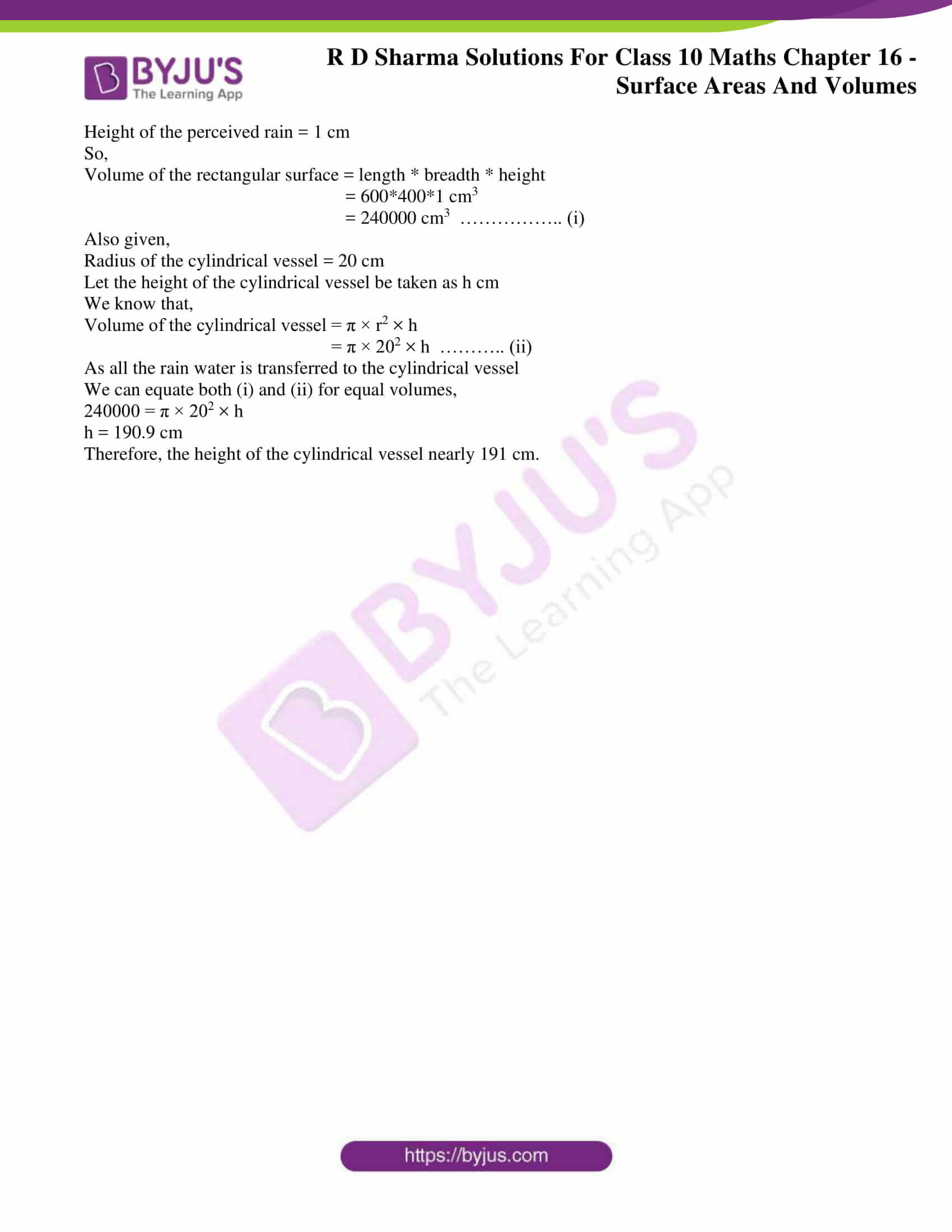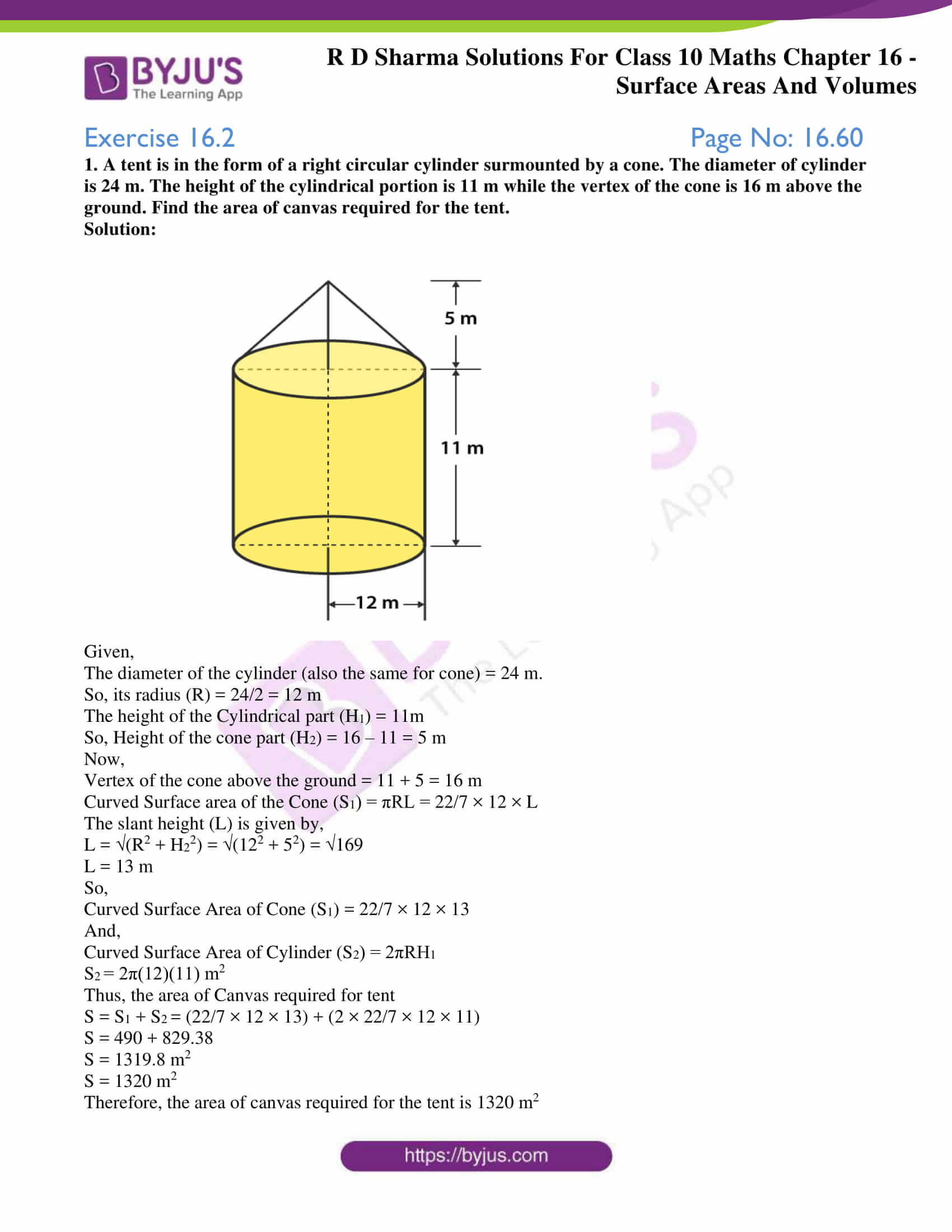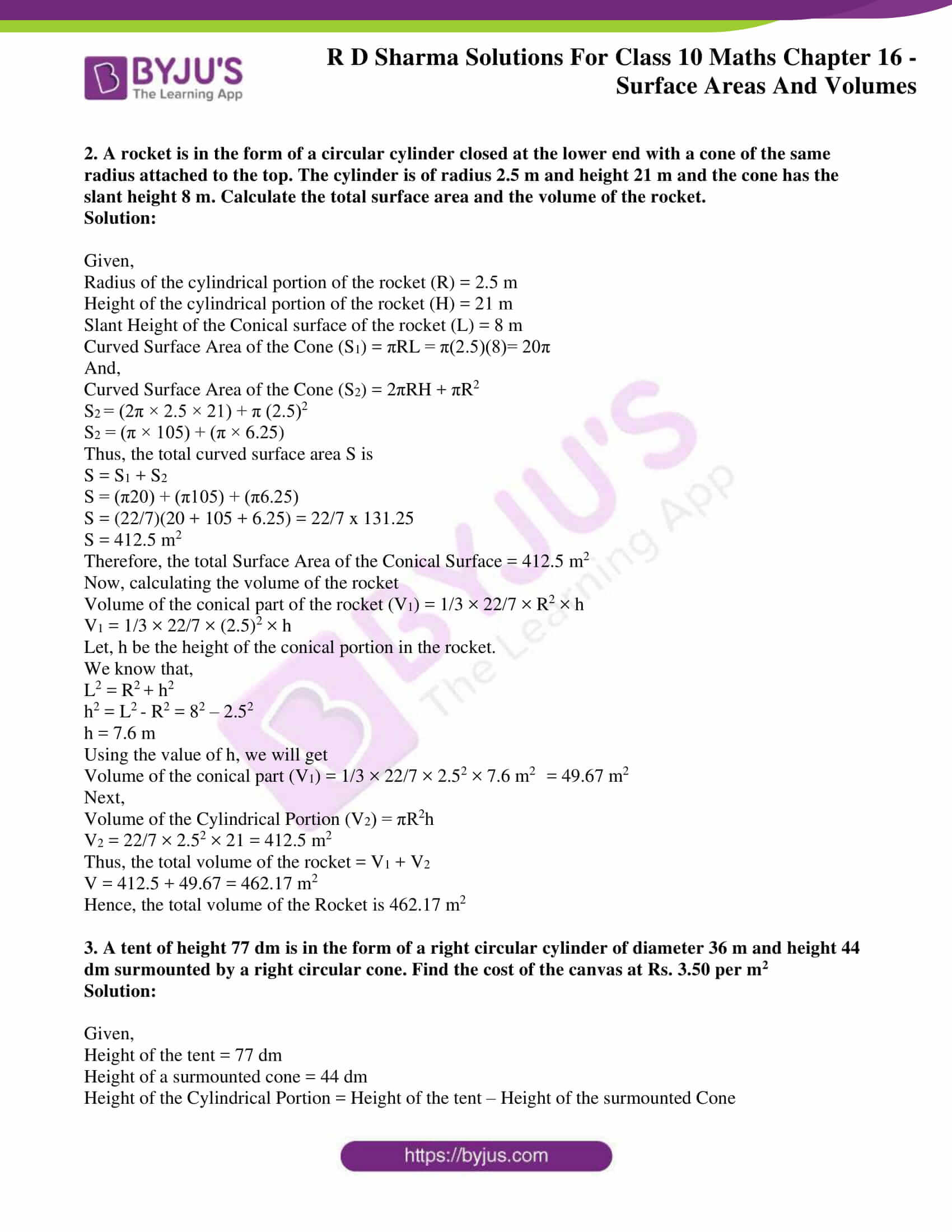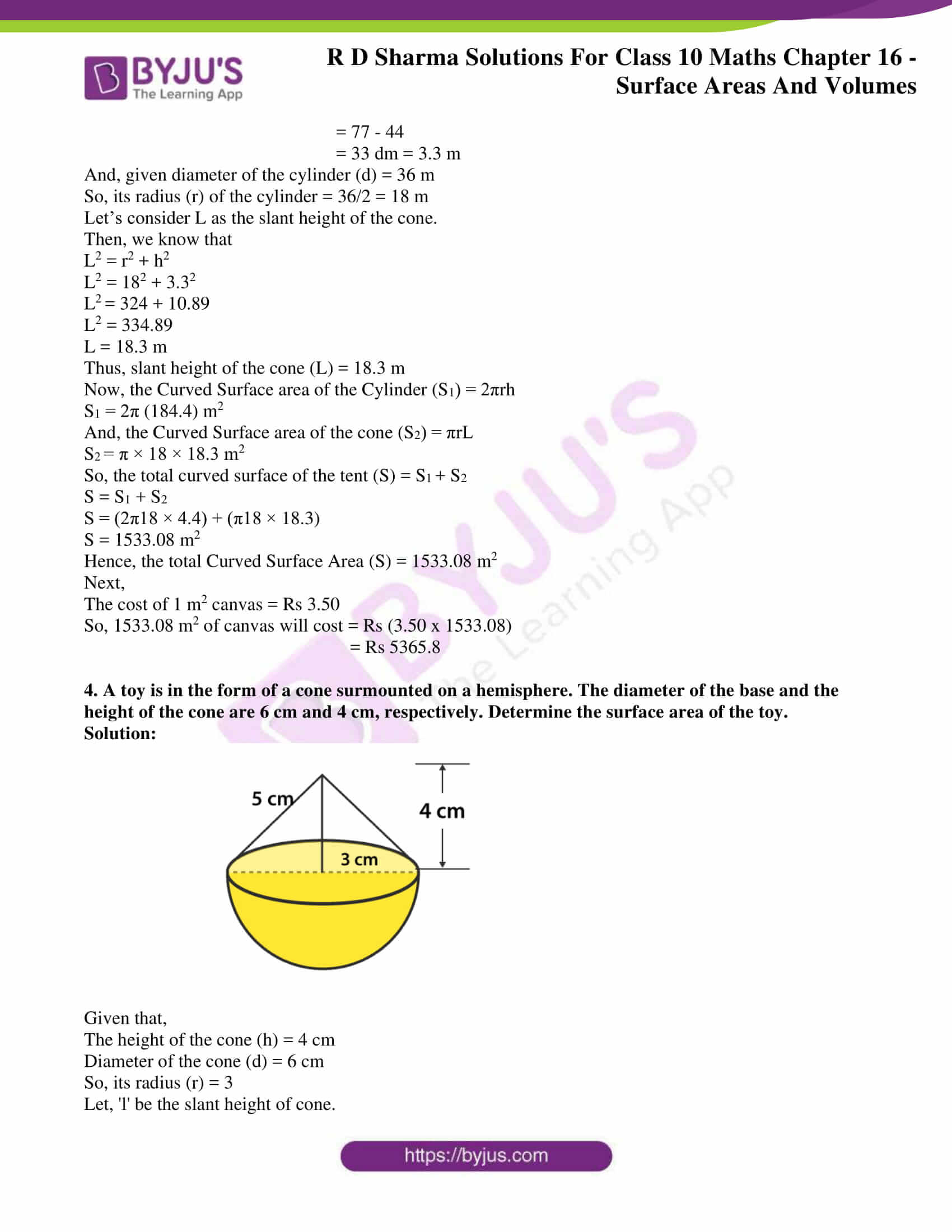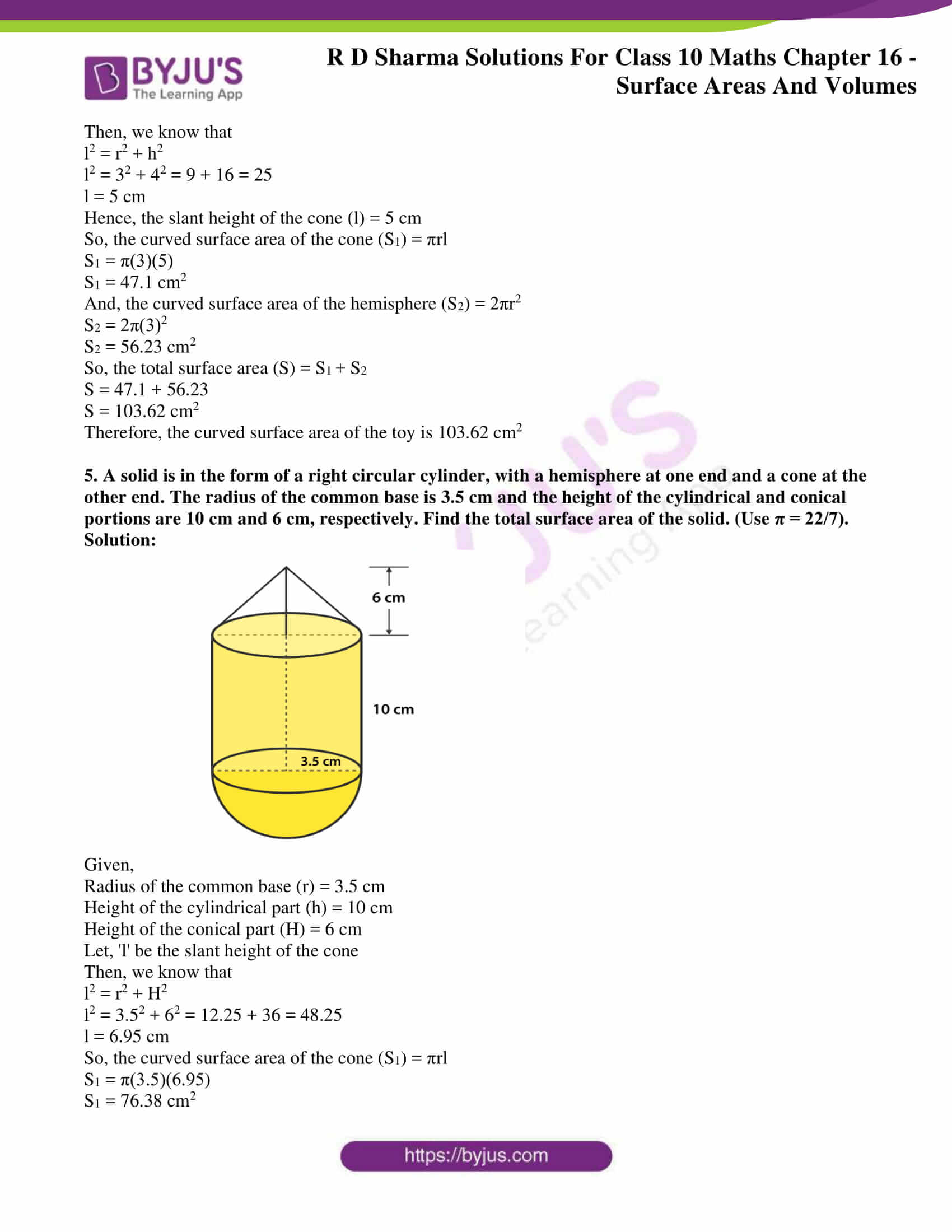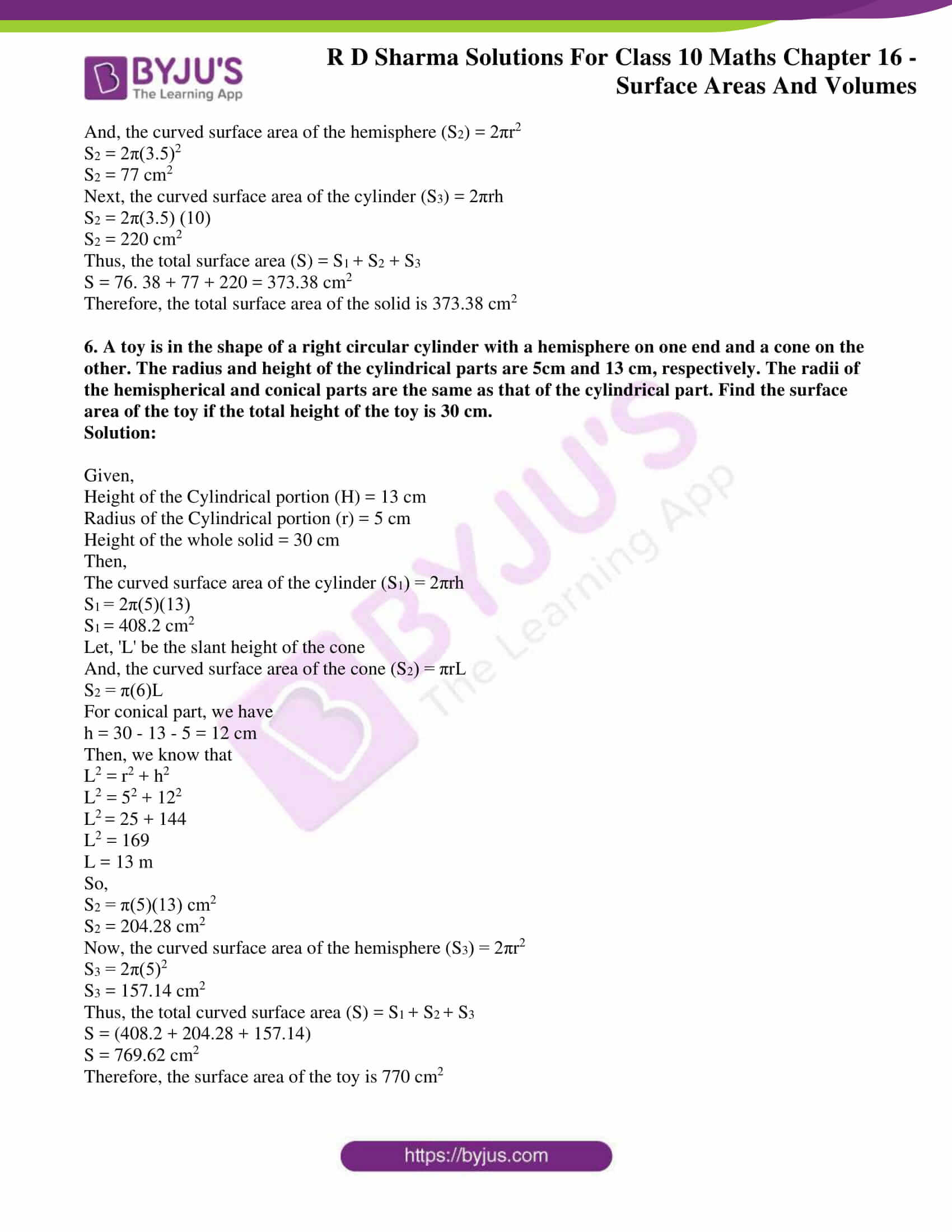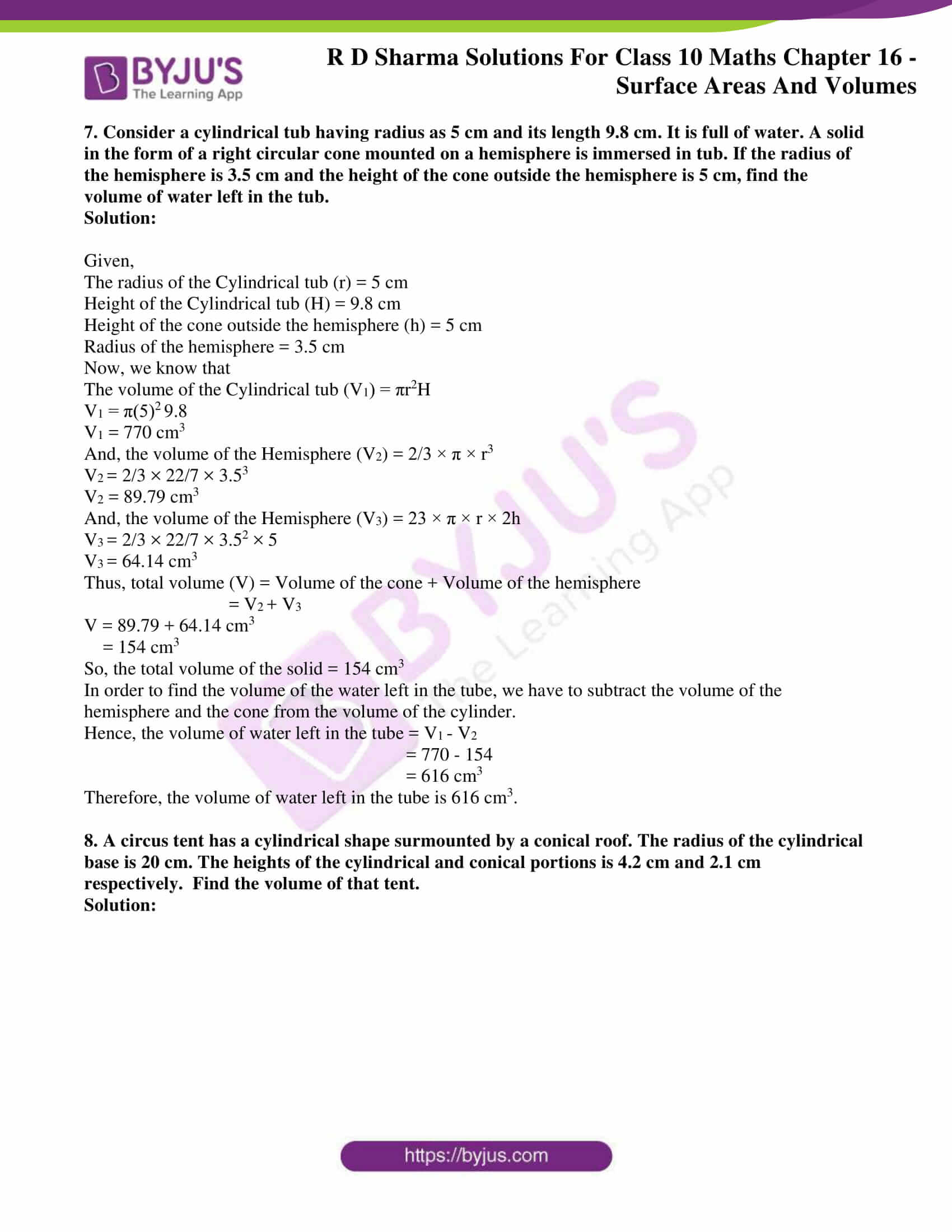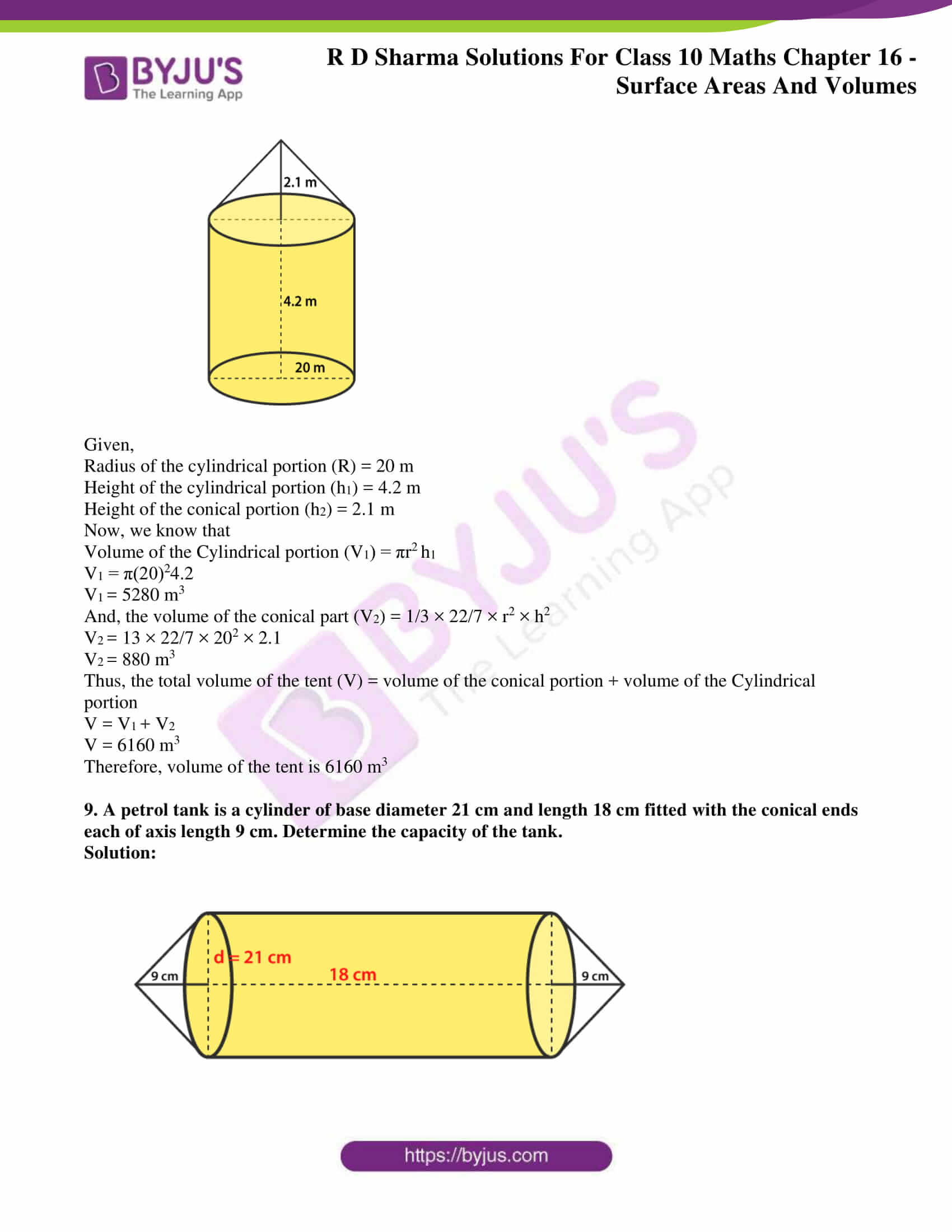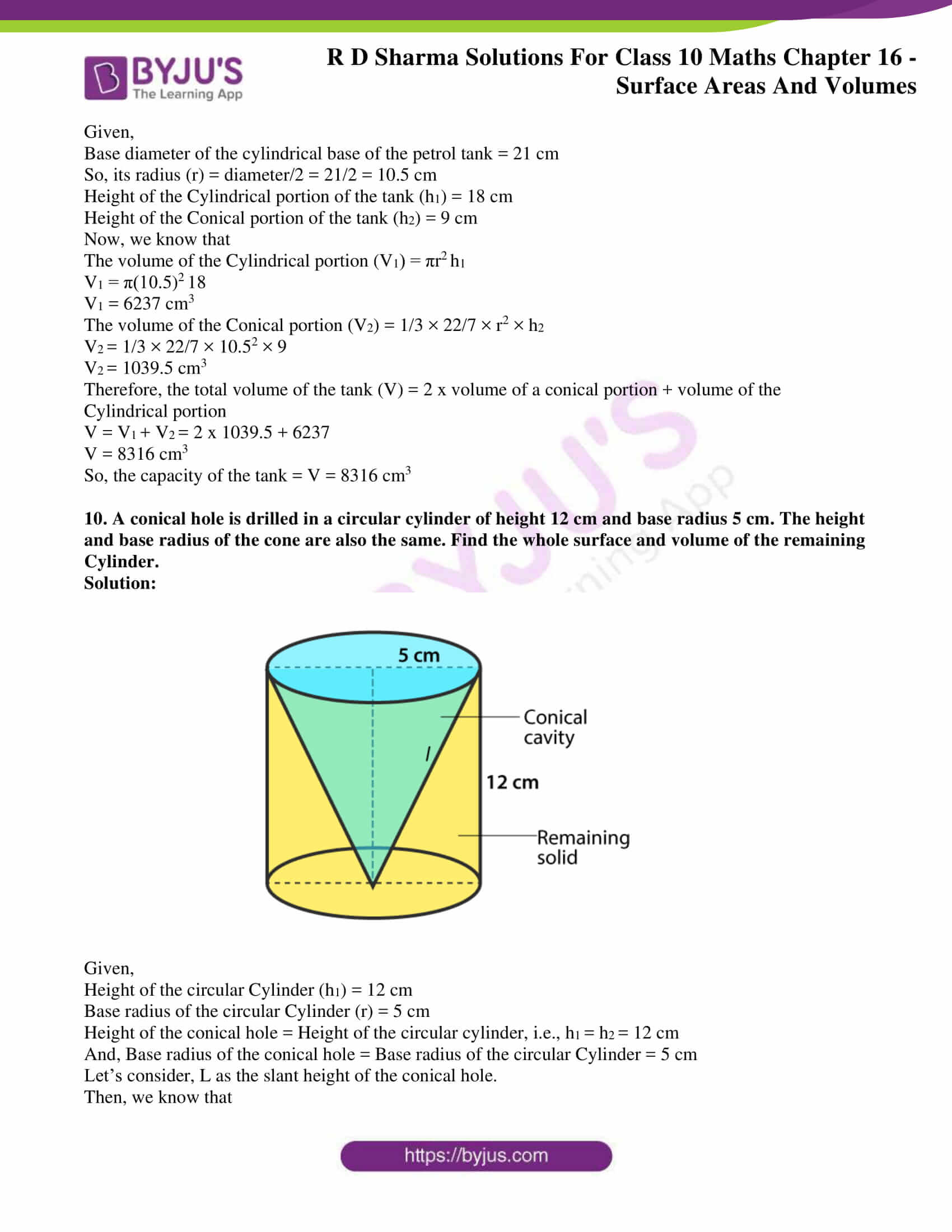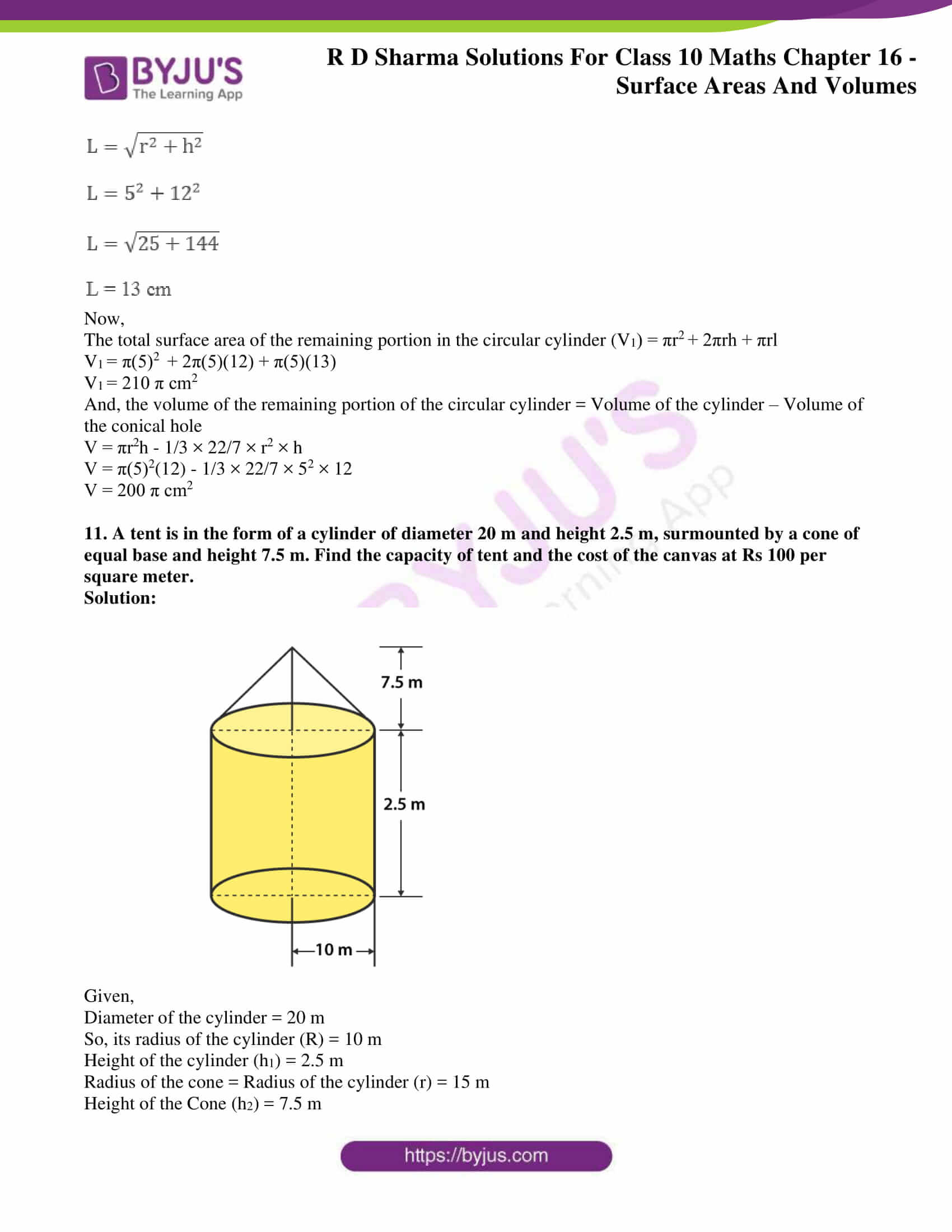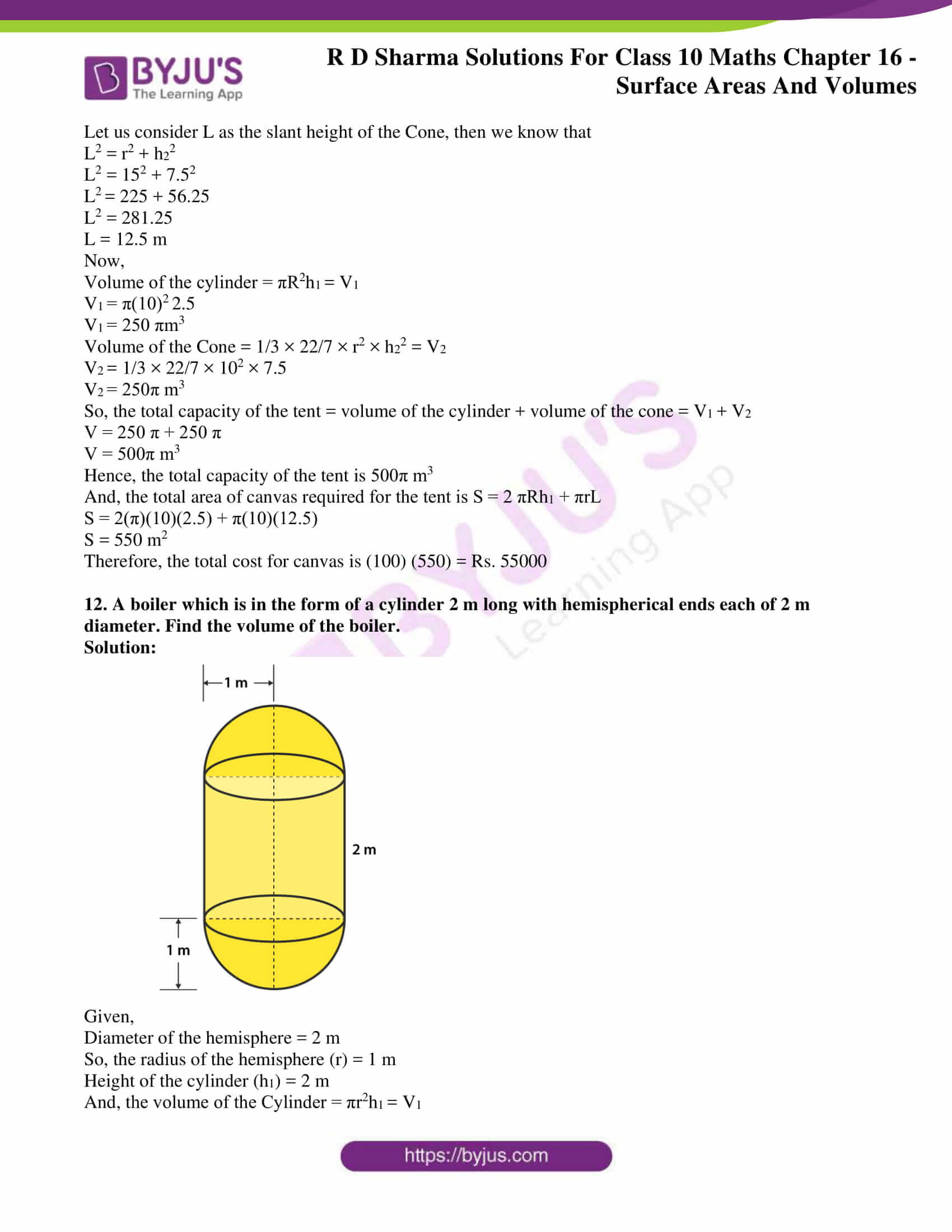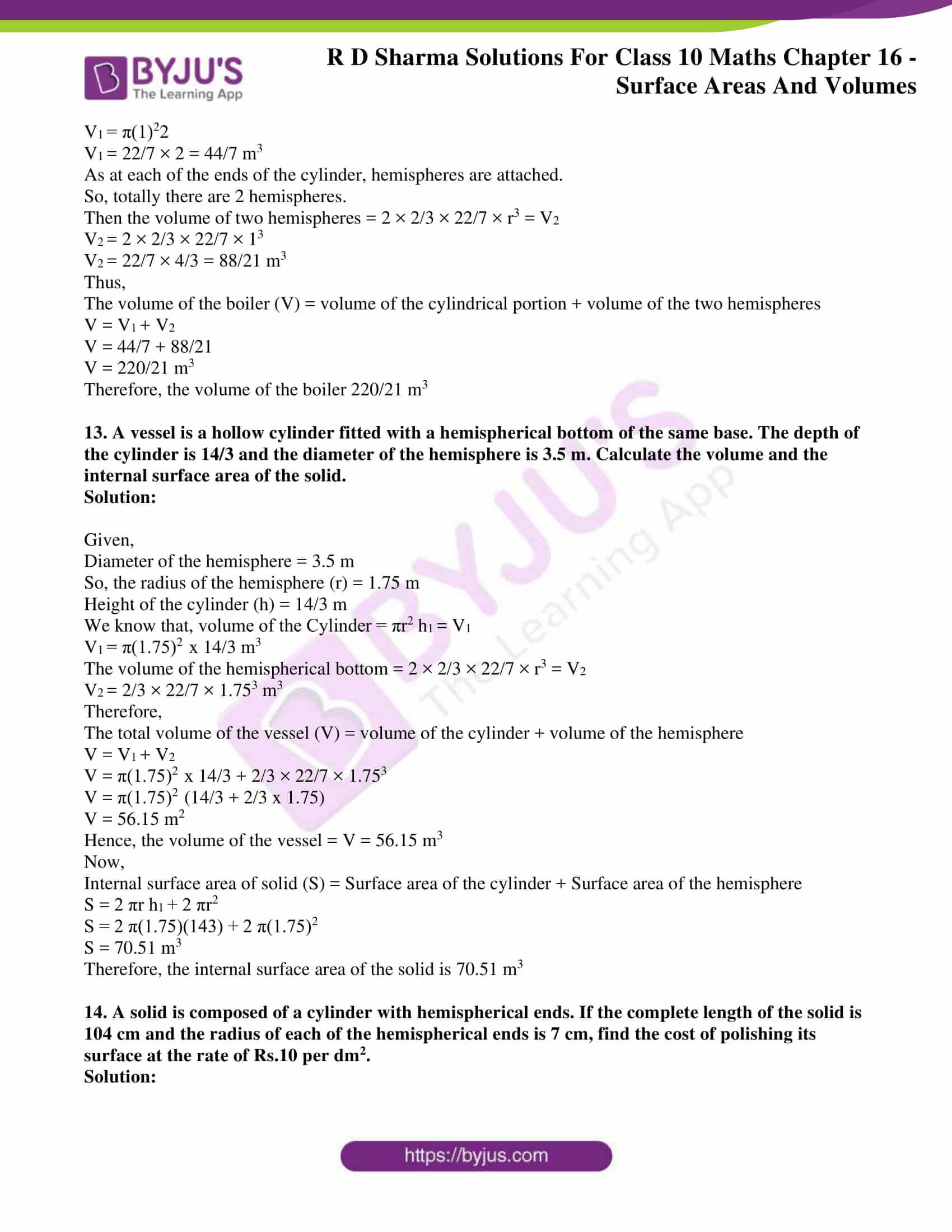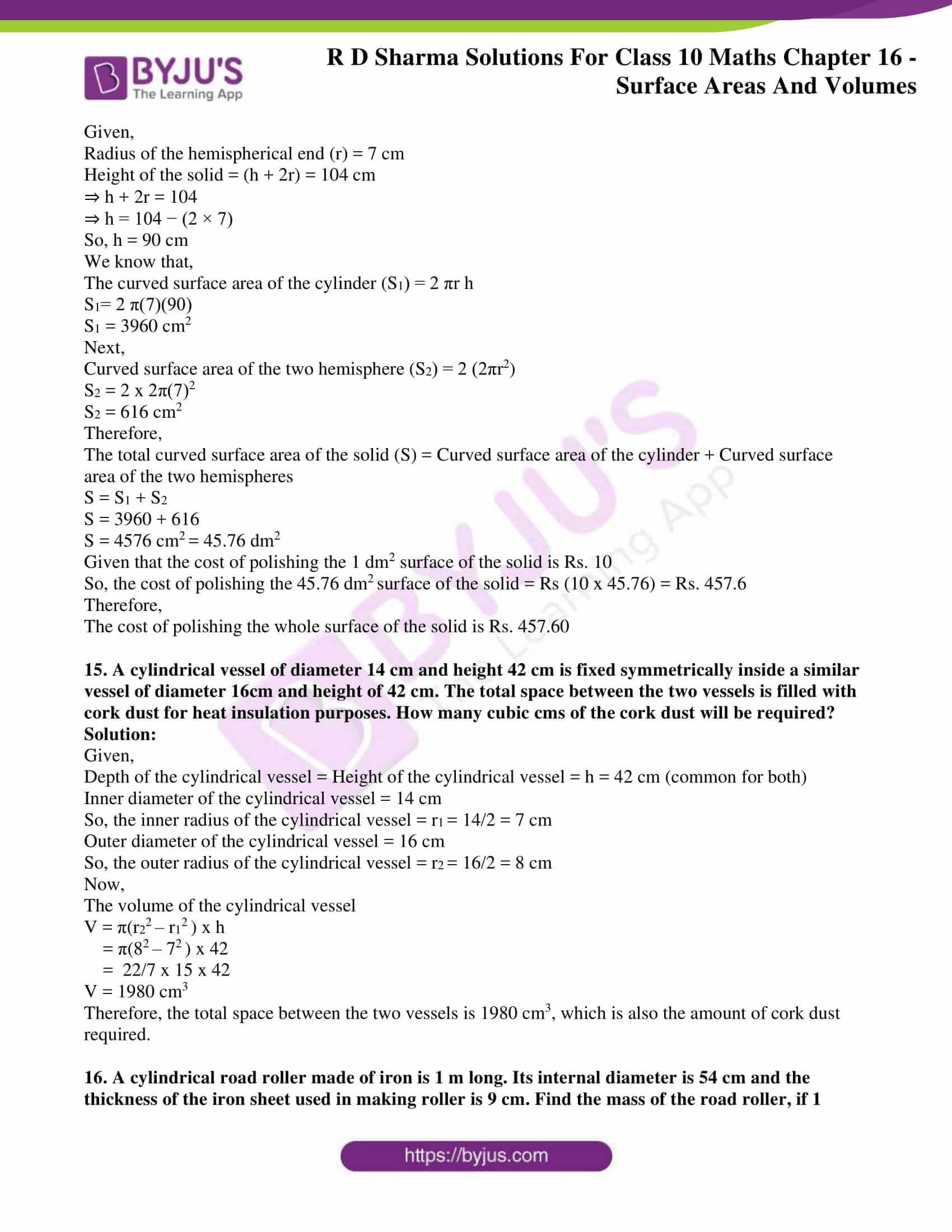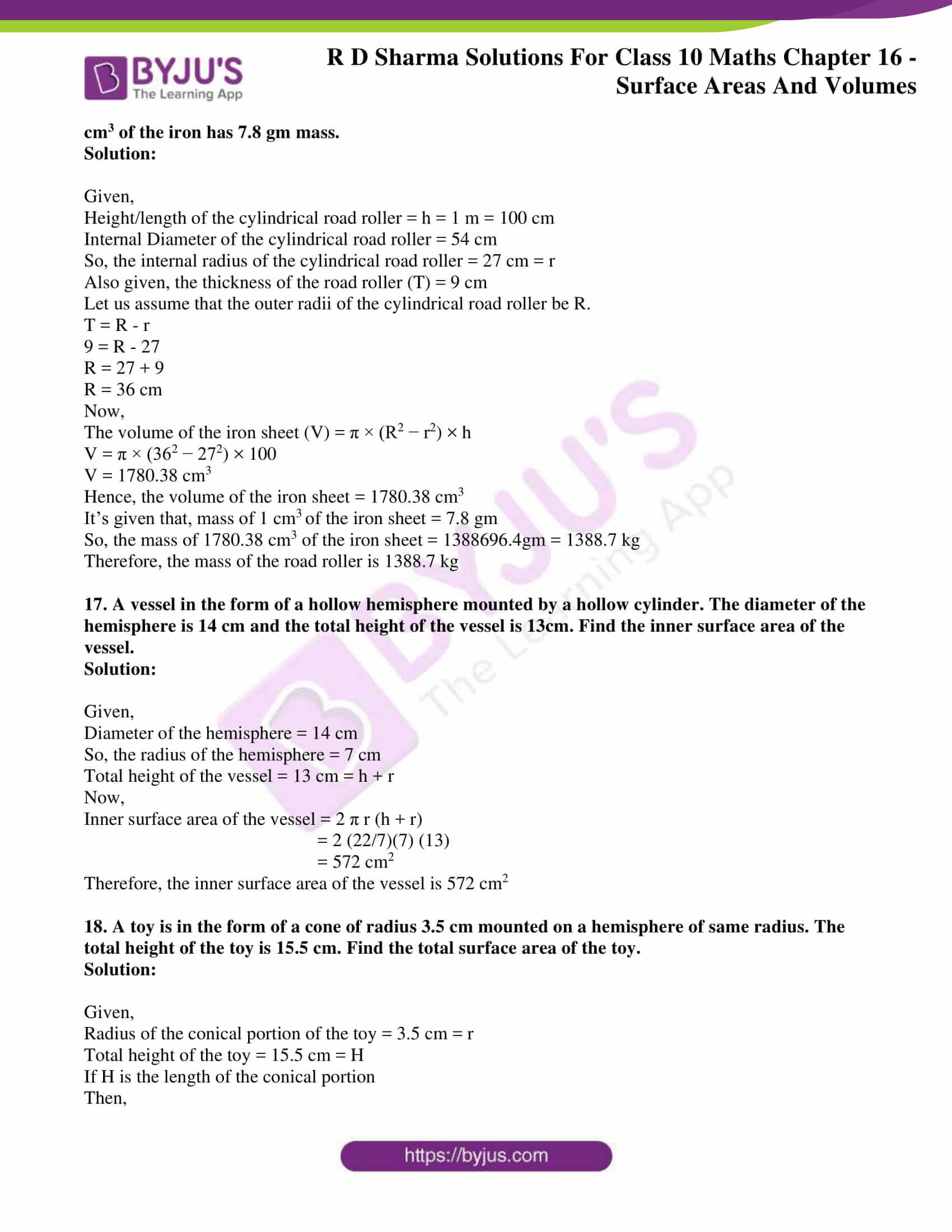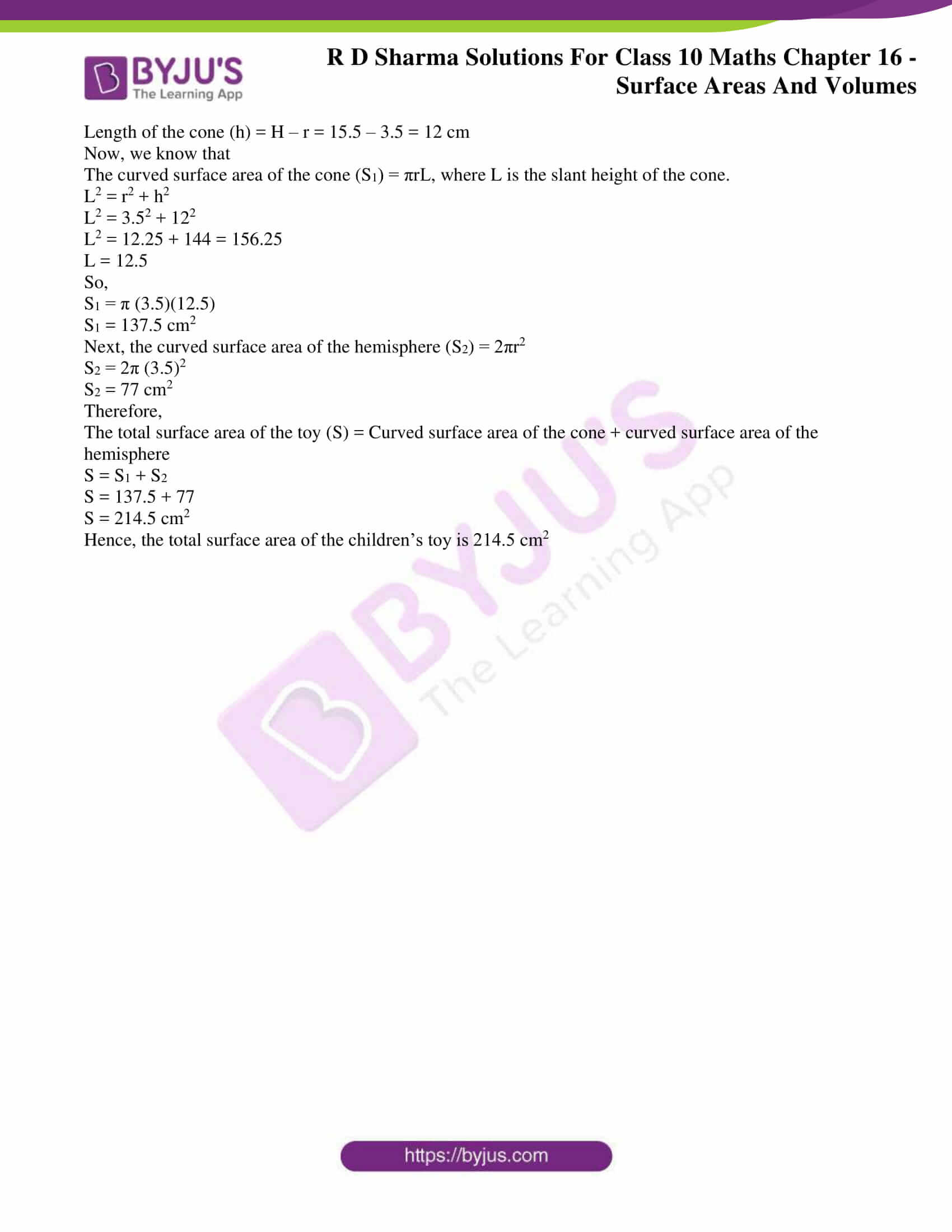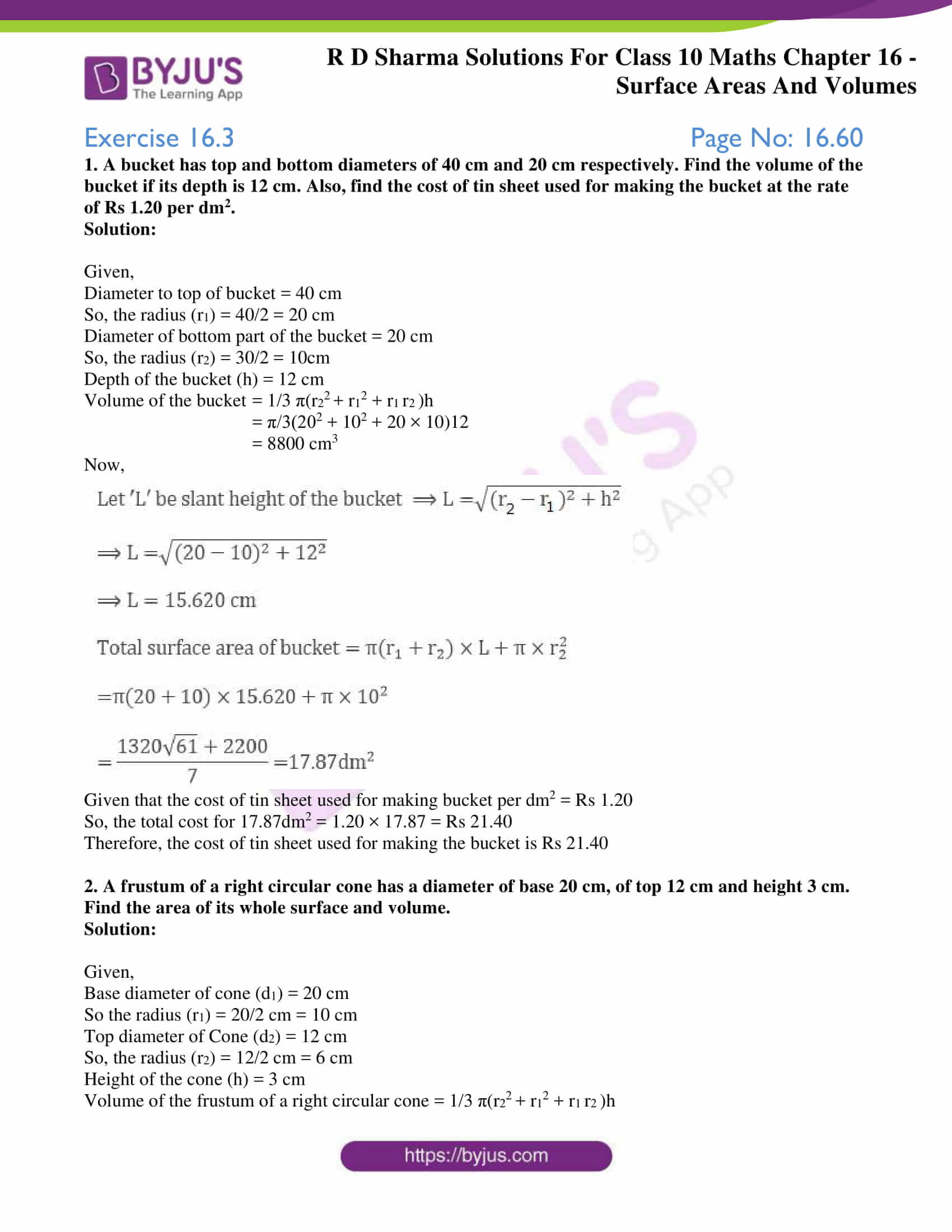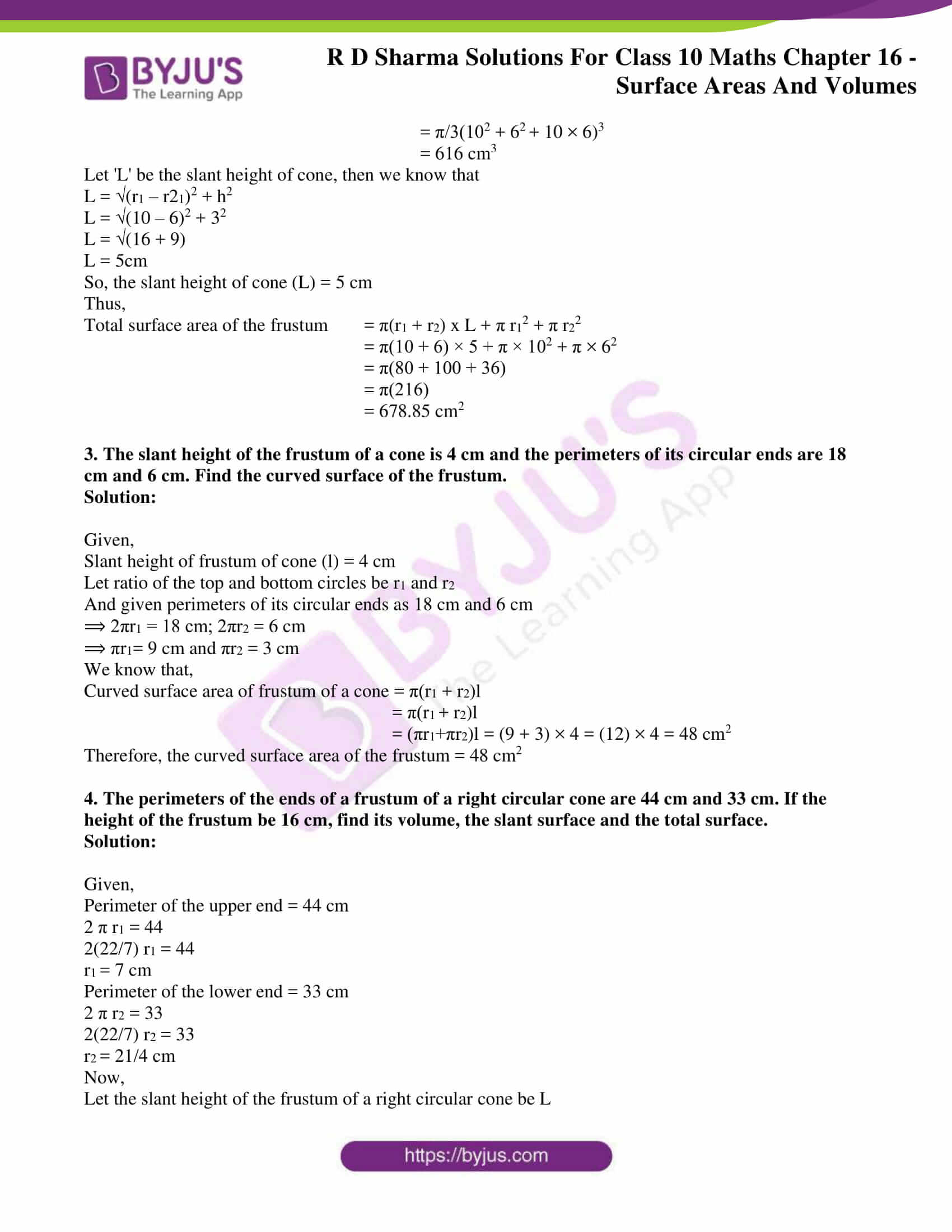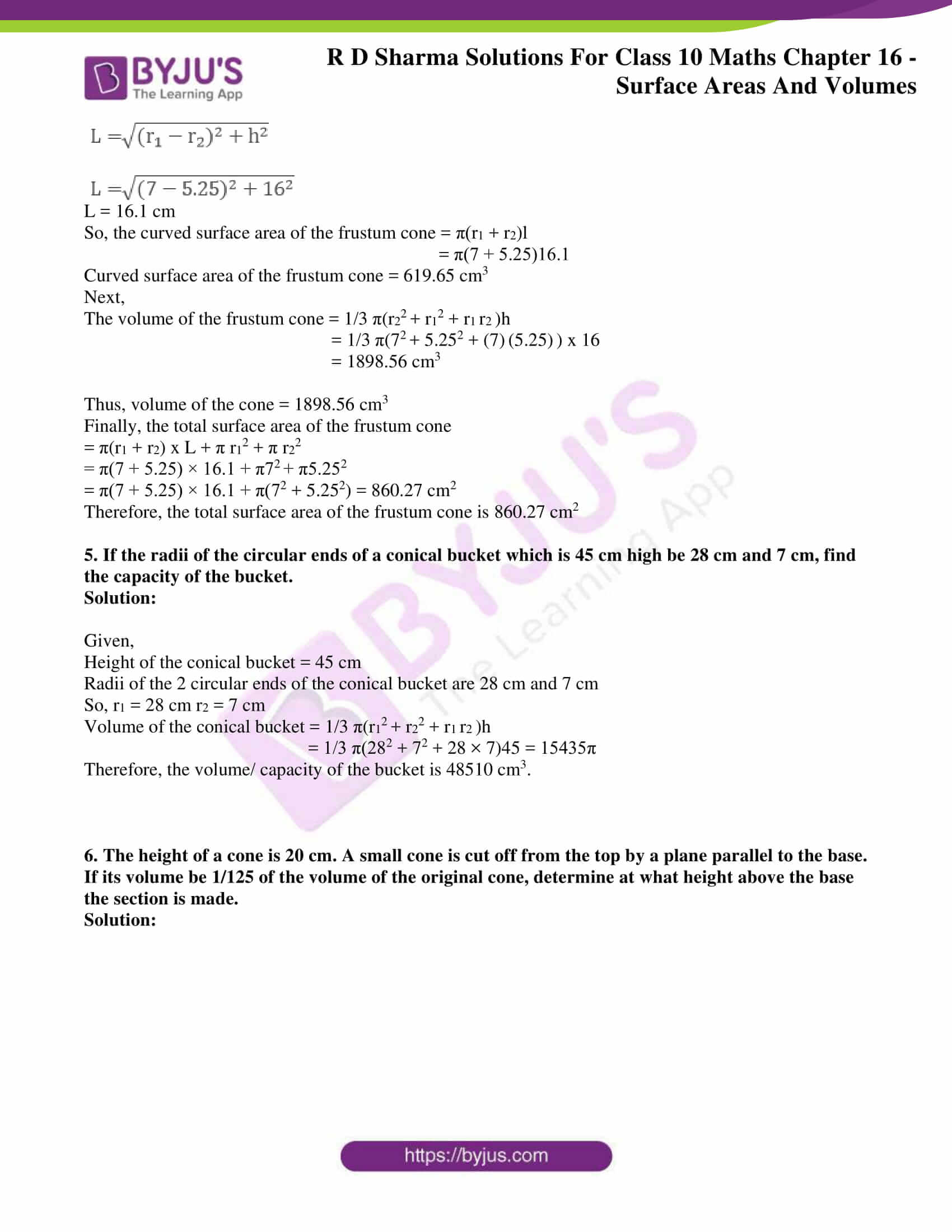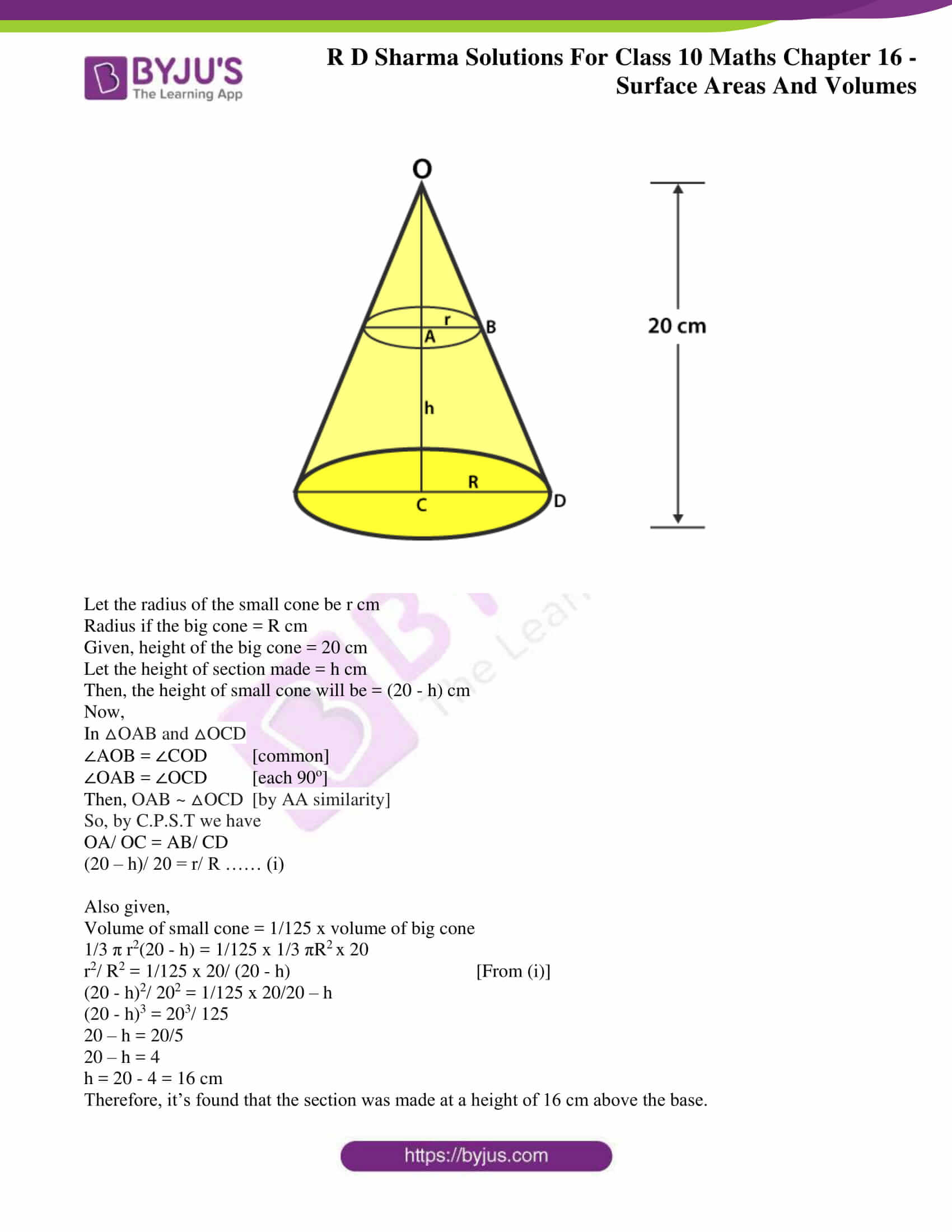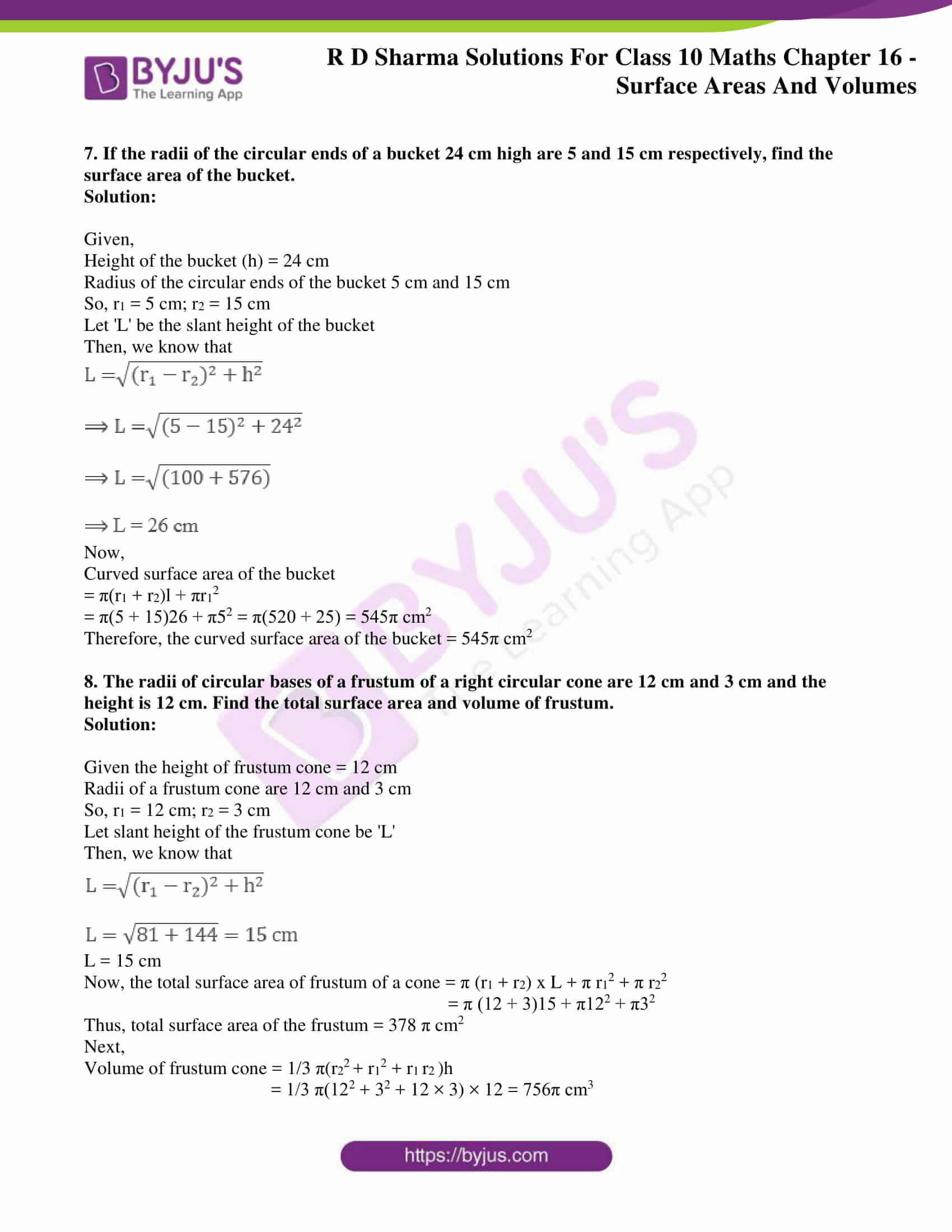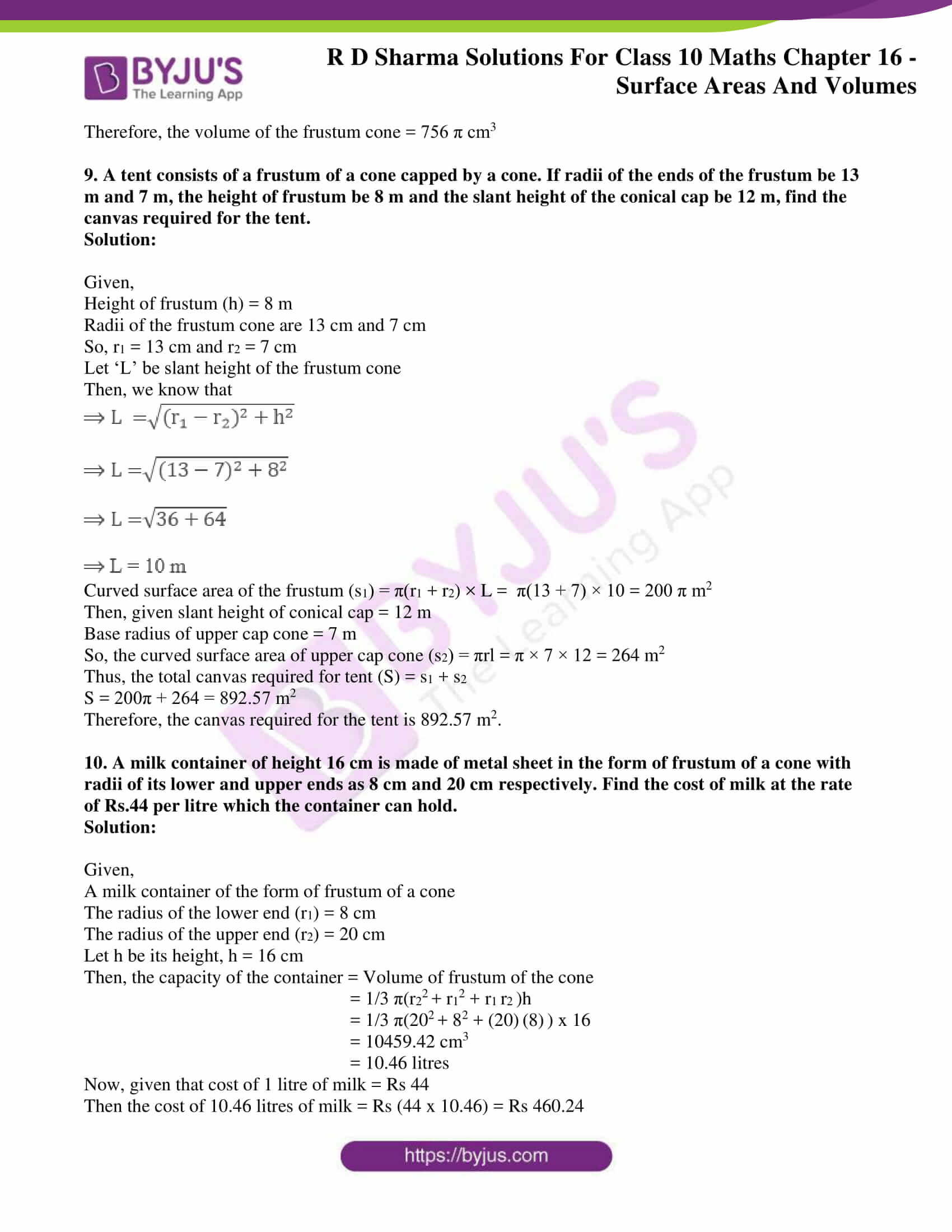### Access the RD Sharma Solutions for Class 10 Maths Chapter 16 – Surface Areas and Volumes

Exercise 16.1 Page No: 16.27

Solution:

Given,

A solid sphere of radius, R = 8 cm

With this sphere, we have to make spherical balls of radius r = 1 cm

Let’s assume that the number of balls made as n.

Then, we know that

Volume of the sphere = 4/3 πr3

The volume of the solid sphere = The sum of the volumes of n spherical balls

n x 4/3 πr3 = 4/3 πR3

n x 4/3 π(1)3 = 4/3 π(8)3

n = 83 = 512

Therefore, 512 balls can be made of radius 1 cm each with a solid sphere of radius 8 cm.

2. How many spherical bullets, each of 5 cm in diameter, can be cast from a rectangular block of metal 11dm x 1 m x 5 dm?

Solution:

Given,

A metallic block of dimension 11dm x 1m x 5dm.

The diameter of each bullet = 5 cm

We know that,

Volume of the sphere = 4/3 πr3

Since, 1 dm = 10-1m = 0.1 m

The volume of the rectangular block = 1.1 x 1 x 0.5 = 0.55 m3

Radius of the bullet = 5/2 = 2.5 cm

Let the number of bullets made from the rectangular block be n.

Then from the question,

The volume of the rectangular block = The sum of the volumes of the n spherical bullets

0.55 = n x 4/3 π(2.5)3

Solving for n, we have

n = 8400

Therefore, 8400 can be cast from the rectangular block of metal.

3. A spherical ball of radius 3 cm is melted and recast into three spherical balls. The radii of the two balls are 2 cm and 1.5 cm, respectively. Determine the diameter of the third ball.

Solution:

Given,

The radius of the spherical ball = 3 cm

We know that,

The volume of the sphere = 4/3 πr3

So, its volume (V) = 4/3 πr3

That the ball is melted and recast into 3 spherical balls.

Volume (V1) of first ball = 4/3 π 1.53

Volume (V2) of second ball = 4/3 π23

Let the radius of the third ball = r cm

Volume of the third ball (V3) = 4/3 πr3

Volume of the spherical ball is equal to the volume of the 3 small spherical balls.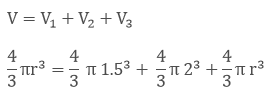Now,

Cancelling out the common part from both sides of the equation, we get

(3)3 = (2)3 + (1.5)+ r3

r= 33– 23– 1.53 cm3

r= 15.6 cm3

r = (15.6)1/3 cm

r = 2.5 cm

As diameter = 2 x radius = 2 x 2.5 cm

= 5.0 cm.

Thus, the diameter of the third ball is 5 cm.

4. 2.2 cubic dm of brass is to be drawn into a cylindrical wire of 0.25 cm in diameter. Find the length of the wire.

Solution:

Given,

2.2 dm3 of brass is to be drawn into a cylindrical wire of Diameter = 0.25 cm

So, the radius of the wire (r) = d/2

= 0.25/2 = 0.125*10-2 cm

Now, 1 cm = 0.01 m

So, 0.1cm = 0.001 m

Let the length of the wire be (h)

We know that,

The volume of the cylinder = πr2h

It’s understood that,

The volume of cylindrical wire = The volume of brass of 2.2 dm3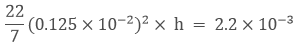h = 448 m

Therefore, the length of the cylindrical wire drawn is 448 m.

5. What length of a solid cylinder 2 cm in diameter must be taken to recast into a hollow cylinder of length 16 cm, external diameter 20 cm and thickness 2.5 mm?

Solution:

Given,

Diameter of the solid cylinder = 2 cm

Length of hollow cylinder = 16 cm

The solid cylinder is recast into a hollow cylinder of length 16 cm, the external diameter of 20 cm and thickness of 2.5 mm = 0.25 cm

We know that,

Volume of a cylinder = πr2h

Radius of the solid cylinder = 1 cm

So,

Volume of the solid cylinder = π12h = πh cm3

Let’s assume the length of the solid cylinder as h.

And,

Volume of the hollow cylinder = πh(R2– r2)

Thickness of the cylinder = (R – r)

0.25 = 10 – r

So, the internal radius of the cylinder is 9.75 cm.

Volume of the hollow cylinder = π × 16 (100 – 95.0625)

Hence, it’s understood that

Volume of the solid cylinder = Volume of the hollow cylinder

πh = π × 16(100 – 95.06)

h = 79.04 cm

Therefore, the length of the solid cylinder is 79.04 cm.

6. A cylindrical vessel having a diameter equal to its height is full of water which is poured into two identical cylindrical vessels with diameter 42 cm and height 21 cm, which are filled completely. Find the diameter of the cylindrical vessel.

Solution:

Given,

The diameter of the cylinder = The height of the cylinder

⇒ h = 2r, where h – the height of the cylinder and r – the radius of the cylinder

We know that,

Volume of a cylinder = πr2h

So, volume of the cylindrical vessel = πr22r = 2πr3   (as h = 2r)….. (i)

Now,

Volume of each identical vessel = πr2h

Diameter = 42 cm, so the radius = 21 cm

Height = 21 cm

So, the volume of two identical vessels = 2 x π 212 × 21 ….. (ii)

Since the volumes on equations (i) and (ii) are equal,

On equating both equations, we have

2πr3= 2 x π 212 × 21

r3 = (21)3

r = 21 cm

So, d = 42 cm

Therefore, the diameter of the cylindrical vessel is 42 cm.

7. 50 circular plates, each of diameter 14 cm and thickness 0.5 cm, are placed one above the other to form a right circular cylinder. Find its total surface area.

Solution:

Given,

50 circular plates, each with diameter 14 cm.

Radius of the circular plates = 7cm

Thickness of the plates = 0.5 cm

As these plates are one above the other, the total thickness of all the plates = 0.5 x 50 = 25 cm

So, the total surface area of the right circular cylinder formed = 2πr × h + 2πr2

= 2πr (h + r)

= 2(22/7) x 7 x (25 + 7)

= 2 x 22 x 32 = 1408 cm2

Therefore, the total surface area of the cylinder is 1408 cm2

8. 25 circular plates, each of radius 10.5 cm and thickness 1.6 cm, are placed one above the other to form a solid circular cylinder. Find the curved surface area and the volume of the cylinder so formed.

Solution:

Given,

250 circular plates, each with radius 10.5 cm and thickness of 1.6 cm.

As the plates are placed one above the other, the total height becomes = 1.6 x 25 = 40 cm

We know that,

Curved surface area of a cylinder = 2πrh

= 2π × 10.5 × 40 = 2640 cm2

And, volume of the cylinder = πr2h

= π × 10.5× 40 = 13860 cm3

Therefore,

The curved surface area of the cylinder is 2640 cm2, and the volume of the cylinder is 13860 cm3

9. Find the number of metallic circular discs with 1.5 cm base diameter and of height 0.2 cm to be melted to form a right circular cylinder of height 10 cm and diameter 4.5 cm.

Solution:

Given,

Radius of each circular disc = r = 1.5/2 = 0.75 cm

Height of each circular disc = h = 0.2 cm

Radius of cylinder = R = 4.5/ 2 = 2.25 cm

Height of cylinder = H = 10 cm

So, the number of metallic discs required is given by n

n = Volume of cylinder / Volume of each circular disc

n = πR2H/ πr2h

n = (2.25)2(10)/ (0.75)2(0.2)

n = 3 x 3 x 50 = 450

Therefore, 450 metallic discs are required.

10. How many spherical lead shots, each of diameter 4.2 cm, can be obtained from a solid rectangular lead piece with dimensions 66 cm × 42 cm × 21 cm?

Solution:

Given,

Radius of each spherical lead shot = r = 4.2/ 2 = 2.1 cm

The dimensions of the rectangular lead piece = 66 cm x 42 cm x 21 cm

So, the volume of a spherical lead shot = 4/3 πr3

= 4/3 x 22/7 x 2.13

And the volume of the rectangular lead piece = 66 x 42 x 21

Thus,

The number of spherical lead shots = Volume of rectangular lead piece/ Volume of a spherical lead shot

= 66 x 42 x 21/ (4/3 x 22/7 x 2.13)

= 1500

11. How many spherical lead shots of diameter 4 cm can be made out of a solid cube of lead whose edge measures 44 cm?

Solution:

Given,

The radius of each spherical lead shot = r = 4/2 = 2 cm

Volume of each spherical lead shot = 4/3 πr3 = 4/3 π 23 cm3

Edge of the cube = 44 cm

Volume of the cube = 443 cm3

Thus,

Number of spherical lead shots = Volume of cube/ Volume of each spherical lead shot

= 44 x 44 x 44/ (4/3 π 23)

= 2541

12. Three cubes of a metal whose edges are in the ratio 3: 4: 5 are melted and converted into a single cube whose diagonal is 12√3 cm. Find the edges of the three cubes.

Solution:

Let the edges of three cubes (in cm) be 3x, 4x and 5x, respectively.

So, the volume of the cube after melting will be = (3x)3 + (4x)3 + (5x)3

= 9x3 + 64x3 + 125x3 = 216x3

Now, let a be the edge of the new cube so formed after melting.

Then we have,

a3 = 216x3

a = 6x

We know that,

Diagonal of the cube = (a2 + a2 + a2) = a3

So, 123 = a3

a = 12 cm

x = 12/6 = 2

Thus, the edges of the three cubes are 6 cm, 8 cm and 10 cm, respectively.

13. A solid metallic sphere of radius 10.5 cm is melted and recast into a number of smaller cones, each of radius 3.5 cm and height 3 cm. Find the number of cones so formed.

Solution:

Given,

Radius of metallic sphere = R = 10.5 cm

So, its volume = 4/3 πR3 = 4/3 π(10.5)3

We also have,

Radius of each cone = r = 3.5 cm

Height of each cone = h = 3 cm

And, its volume = 1/3 πr2h = 1/3 π(3.5)2(3)

Thus,

The number of cones = Volume of metallic sphere/ Volume of each cone

= 4/3 π(10.5)3 / 1/3 π(3.5)2(3)

= 126

14. The diameter of a metallic sphere is equal to 9 cm. It is melted and drawn into a long wire of diameter 2 mm having a uniform cross-section. Find the length of the wire.

Solution:

Given,

Radius of the sphere = 9/2 cm

So, its volume = 4/3 πr3 = 4/3 π(9/2)3

And, the radius of the wire = 2 mm = 0.2 cm

Let the length of the wire = h cm

So, the volume of wire = πr2h = π(0.2)2h

Then, according to the question we have

Volume of wire = Volume of sphere

π(0.2)2h = 4/3 π(9/2)3

h = 4 x 729/ (3 x 8 x 0.01) = 12150 cm

Therefore, the length of the wire = 12150 cm

15. An iron spherical ball has been melted and recast into smaller balls of equal size. If the radius of each of the smaller balls is 1/4 of the radius of the original ball, how many such balls are made? Compare the surface area of all the smaller balls combined together with that of the original ball.

Solution:

Let the radius of the big ball be x cm

The radius of the small ball = x/4 cm

And, let the number of balls = n

Then according to the question, we have

Volume of n small balls = Volume of the big ball

n x 4/3 π(x/4)3 = 4/3 πx3

n x (x3/ 64) = x3

n = 64

Therefore, the number of small balls = 64

Next,

Surface area of all small balls/ Surface area of big ball = 64 x 4π(x/4)2/ 4π(x)2

= 64/16 = 4/1

Thus, the ratio of the surface area of the small balls to that of the original ball is 4:1.

16. A copper sphere of radius 3 cm is melted and recast into a right circular cone of height 3 cm. Find the radius of the base of the cone.

Solution:

Given,

Radius of the copper sphere = 3 cm

We know that,

Volume of the sphere = 4/3 π r3

= 4/3 π × 33 ….. (i)

Also, given that the copper sphere is melted and recasted into a right circular cone.

Height of the cone = 3 cm

We know that,

Volume of the right circular cone = 1/3 π r2h

= 1/3 π × r× 3 ….. (ii)

On comparing equations (i) and (ii), we have

4/3 π × 33 = 1/3 π × r× 3

r2 = 36

r = 6 cm

Therefore, the radius of the base of the cone is 6 cm.

17. A copper rod of diameter 1 cm and length 8 cm is drawn into a wire of length 18 m of uniform thickness. Find the thickness of the wire.

Solution:

Given,

Diameter of the copper wire = 1 cm

So, radius of the copper wire = 1/2 cm = 0.5 cm

Length of the copper rod = 8 cm

We know that,

Volume of the cylinder = π r2h

= π × 0.5× 8 ……. (i)

Length of the wire = 18 m = 1800 cm

Volume of the wire = π r2h

= π r2 × 1800  ….. (ii)

On equating both equations, we have

π × 0.5× 8 = π r2 × 1800

r2 = 2 /1800 = 1/900

r = 1/30 cm

Therefore, the diameter of the wire is 1/15 cm, i.e., 0.67 mm, which is the thickness of the wire.

18. The diameters of the internal and external surfaces of a hollow spherical shell are 10cm and 6 cm, respectively. If it is melted and recast into a solid cylinder of the length of 8/3, find the diameter of the cylinder.

Solution:

Given,

The internal diameter of the hollow sphere = 6 cm

So, the internal radius of the hollow sphere = 6/2 cm = 3 cm = r

The external diameter of the hollow sphere = 10 cm

So, the external radius of the hollow sphere = 10/2 cm = 5 cm = R

We know that,

Volume of the hollow spherical shell = 4/3 π × (R3 – r3)

= 4/3 π × (53 – 33)         …..  (i)

And given, the length of the solid cylinder = 8/3 cm

Let the radius of the solid cylinder be r cm.

We know that,

Volume of the cylinder = π × r2 × h

= π × r2 × 8/3       ….. (ii)

Now equating both (i) and (ii), we have

4/3 π × 53 – 33 = π × r2 × 8/3

4/3 x (125 – 27) = r2 × 8/3

98/2 = r2

r2 = 49

r = 7

So, d = 7 x 2 = 14 cm

Therefore, the diameter of the cylinder is 14 cm.

19. How many coins 1.75 cm in diameter and 2 mm thick must be melted to form a cuboid 11 cm x 10 cm x 7 cm?

Solution:

Given,

Diameter of the coin = 1.75 cm

So, its radius = 1.74/2 = 0.875 cm

Thickness or the height = 2 mm = 0.2 cm

We know that,

Volume of the cylinder (V1) = πr2h

= π 0.8752 × 0.2

And, the volume of the cuboid (V2) = 11 × 10 × 7 cm3

Let the number of coins needed to be melted be n.

So, we have

V2 = V1 × n

11 × 10 × 7 = π 0.8752 × 0.2 x n

11 × 10 × 7 = 22/7 x 0.8752 × 0.2 x n

On solving, we get n = 1600

Therefore, the number of coins required is 1600.

20. The surface area of a solid metallic sphere is 616 cm2. It is melted and recast into a cone of height 28 cm. Find the diameter of the base of the cone so formed.

Solution:

Given,

The height of the cone = 28 cm

Surface area of the solid metallic sphere = 616 cm3

We know that,

Surface area of the sphere = 4πr2

So, 4πr2 = 616

r2 = 49

r = 7

Radius of the solid metallic sphere = 7 cm

Let’s assume r to be the radius of the cone.

We know that,

Volume of the cone = 1/3 πr2h

= 1/3 πr(28) ….. (i)

Volume of the sphere = 4/3 πr3

= 4/3 π73  ……….  (ii)

On equating equations (i) and (ii), we have

1/3 πr(28) = 4/3 π73

Eliminating the common terms, we get

r(28) = 4 x 73

r= 49

r =7

So, diameter of the cone = 7 x 2 = 14 cm

Therefore, the diameter of the base of the cone is 14 cm.

21. A cylindrical bucket, 32 cm high and 18 cm of radius of the base, is filled with sand. This bucket is emptied on the ground, and a conical heap of sand is formed. If the height of the conical heap is 24 cm, find the radius and slant height of the heap.

Solution:

Given,

Height of the cylindrical bucket = 32 cm

Radius of the cylindrical bucket = 18 cm

Height of the conical heap = 24 cm

We know that,

Volume of the cylinder = π × r2 × h

And, volume of the cone = 1/3 π × r2 × h

Then, from the question,

Volume of the conical heap = Volume of the cylindrical bucket

1/3 π × r2 × 24 = π × 182 × 32

r2 = 182 x 4

r = 18 x 2 = 36 cm

Now,

The slant height of the conical heap (l) is given by

l = √(h2 + r2)

l = √(242 + 362) = √1872

l = 43.26 cm

Therefore, the radius and slant height of the conical heap are 36 cm and 43.26 cm, respectively.

22. A solid metallic sphere of radius 5.6 cm is melted, and solid cones, each of radius 2.8 cm and height 3.2 cm, are made. Find the number of such cones formed.

Solution:

Let the number of cones made be n

Given,

Radius of metallic sphere = 5.6 cm

Radius of the cone = 2.8 cm

Height of the cone = 3.2 cm

We know that,

Volume of a sphere = 4/3 π × r3

So, V1 = 4/3 π × 5.63

And,

Volume of cone = 1/3 π × r2 × h

V2 = 1/3 π × 2.82 × 3.2

Thus, the number of cones (n) = Volume of the sphere/ Volume of the cone

n = 4/3 π × 5.63 / (1/3 π × 2.82 × 3.2)

n = (4 x 5.63)/ (2.82 × 3.2)

n = 28

Therefore, 28 such cones can be formed.

23. A solid cuboid of iron with dimensions 53 cm x 40 cm x 15 cm is melted and recast into a cylindrical pipe. The outer and inner diameters of the pipe are 8 cm and 7 cm, respectively. Find the length of the pipe.

Solution:

Let the length of the pipe be h cm.

Then, volume of the cuboid = (53 x 40 x 15) cm3

Internal radius of the pipe = 7/2 cm = r

External radius of the pipe = 8/2 = 4 cm = R

So, the volume of iron in the pipe = (External Volume) – (Internal Volume)

= πR2h – πr2h

= πh(R2– r2)

= πh(R – r) (R + r)

= π(4 – 7/2) (4 + 7/2) x h

= π(1/2) (15/2) x h

Then from the question, it’s understood that

The volume of iron in the pipe = The volume of iron in the cuboid

π(1/2) (15/2) x h = 53 x 40 x 15

h = (53 x 40 x 15 x 7/22 x 2/15 x 2) cm

h = 2698 cm

Therefore, the length of the pipe is 2698 cm.

24. The diameters of the internal and external surfaces of a hollow spherical shell are 6 cm and 10 cm, respectively. If it is melted and recast into a solid cylinder of diameter 14 cm, find the height of the cylinder.

Solution:

Given,

The internal diameter of the hollow spherical shell = 6 cm

So, the internal radius of the hollow spherical shell = 6/2 = 3 cm = r

The external diameter of hollow spherical shell = 10 cm

So, the external diameter of the hollow spherical shell = 10/2 = 5 cm = R

Diameter of the cylinder = 14 cm

So, the radius of cylinder = 14/2 = 7 cm

Let the height of the cylinder be taken as h cm.

Then, according to the question we have

Volume of cylinder = Volume of spherical shell

π × r2 × h = 4/3 π × (R3 – r3)

π × 72 × h = 4/3 π × (53 – 33)

h = 4/3 x 2

h = 8/3 cm

Therefore, the height of the cylinder = 8/3 cm

25. A hollow sphere of internal and external diameters 4 cm and 8 cm, respectively, is melted into a cone of base diameter 8 cm. Calculate the height of the cone.

Solution:

Given,

The internal diameter of the hollow sphere = 4 cm

So, the internal radius of the hollow sphere = 2 cm

The external diameter of the hollow sphere = 8 cm

So, the external radius of the hollow sphere = 4 cm

We know that,

Volume of the hollow sphere 4/3 π × (43 – 23)          … (i)

Also given,

Diameter of the cone = 8 cm

So, the radius of the cone = 4 cm

Let the height of the cone be x cm.

Volume of the cone 1/3 π × 42 × h          ….. (ii)

As the volume of the hollow sphere and cone are equal. We can equate equations (i) and (ii).

So, we get

4/3 π × (43 – 23) = 1/3 π × 42 × h

4 x (64 – 8) = 16 x h

h = 14

Therefore, the height of the cone so obtained will have a height of 14 cm.

26. A hollow sphere of internal and external radii 2 cm and 4 cm, respectively, is melted into a cone of base radius 4 cm. Find the height and slant height of the cone.

Solution:

Given,

The internal radius of the hollow sphere = 2 cm

The external radius of the hollow sphere = 4 cm

We know that,

Volume of the hollow sphere 4/3 π × (43 – 23)          … (i)

Also given,

The base radius of the cone = 4 cm

Let the height of the cone be x cm

Volume of the cone 1/3 π × 42 × h          ….. (ii)

As the volume of the hollow sphere and cone are equal. We can equate equations (i) and (ii).

So, we get

4/3 π × (43 – 23) = 1/3 π × 42 × h

4 x (64 – 8) = 16 x h

h = 14

Now,

The slant height of the cone (l) is given by

l = √(h2 + r2)

l = √(142 + 42) = √212

l = 14.56 cm

Therefore, the height and slant height of the conical heap are 14 cm and 14.56 cm, respectively.

27. A spherical ball of radius 3 cm is melted and recast into three spherical balls. The radii of two of the balls are 1.5 cm and 2 cm. Find the diameter of the third ball.

Solution:

Given,

Radius of the spherical ball = 3 cm

We know that,

The volume of the sphere = 4/3 πr3

So, its volume (V) = 4/3 πr3

That the ball is melted and recast into 3 spherical balls.

Volume (V1) of first ball = 4/3 π 1.53

Volume (V2) of second ball = 4/3 π23

Let the radius of the third ball = r cm

Volume of the third ball (V3) = 4/3 πr3

Volume of the spherical ball is equal to the volume of the 3 small spherical balls.Now,

Cancelling out the common part from both sides of the equation, we get,

(3)3 = (2)3 + (1.5)+ r3

r= 33– 23– 1.53 cm3

r= 15.6 cm3

r = (15.6)1/3 cm

r = 2.5 cm

As diameter = 2 x radius = 2 x 2.5 cm

= 5.0 cm.

Thus, the diameter of the third ball is 5 cm

28. A path 2 m wide surrounds a circular pond of diameter 40 m. How many cubic meters of gravel is required to grave the path to a depth of 20 cm?

Solution:

Given,

Diameter of the circular pond = 40 m

So, the radius of the pond = 40/2 = 20 m = r

Thickness (width of the path) = 2 m

The whole view of the pond looks like a hollow cylinder.

And the height will be 20 cm = 0.2 m

So,

Thickness (t) = R – r

2 = R – 20

R = 22 m

Volume of the hollow cylinder = π (R2– r2) × h

= π (222– 202) × 0.2

= 52.8 m3

Therefore, 52.8 cubic meters of gravel are required to grave the path to a depth of 20 cm.

29. A 16 m deep well with diameter 3.5 m is dug up, and the earth from it is spread evenly to form a platform 27.5 m by 7m. Find the height of the platform.

Solution:

Let us assume the well to be a solid right-circular cylinder.

Radius(r) of the cylinder = 3.5/2 m = 1.75 m

Depth of the well or height of the cylinder (h) = 16 m

We know that,

Volume of the cylinder (V1) = πr2h

= π × 1.752 × 16

Given,

The length of the platform (l) = 27.5 m

Breadth of the platform (b) =7 m

Now, let the height of the platform be x m.

We know that,

Volume of the rectangle = l*b*h

V2 = 27.5*7*x

The earth dug up is spread evenly to form the platform.

Volumes of both the well and the platform should be the same.

V1 = V2

π × 1.75 × 1.75 × 16 = 27.5 × 7 × x

x = 0.8 m = 80 cm

Therefore, the height of the platform is 80 cm.

30. A well of diameter 2 m is dug 14 m deep. The earth taken out of it is evenly spread all around it to form an embankment of height 40 cm. Find the width of the embankment.

Solution:

Given,

Radius of the circular cylinder (r) = 2/2 m = 1 m

Height of the well (h) = 14 m

We know that,

Volume of the solid circular cylinder = π r2h

= π × 12× 14 …. (i)

And,

The height of the embankment (h) = 40 cm = 0.4 m

Let the width of the embankment be (x) m.

The embankment is a hollow cylinder with an external radius = 1 + x and an internal radius = 1

Volume of the embankment = π × r× h

= π × [(1 + x)2 – (1)2]× 0.4 ….. (ii)

As the well is spread evenly to form an embankment, then the volumes will be the same.

So, on equating equations (i) and (ii), we get

π × 12 × 14 = π × [(1 + x)2 – (1)2] x 0.4

14/0.4 = 1 + x2 + 2x – 1

35 = x2 + 2x

x2 + 2x – 35 = 0

Solving by factorisation method, we have

(x + 7) (x – 5) = 0

So, x = 5 m can only be the solution as it’s a positive value.

Therefore, the width of the embankment is 5 m.

31. A well with an inner radius 4 m is dug up and 14 m deep. Earth taken out of it has spread evenly all around a width of 3 m to form an embankment. Find the height of the embankment.

Solution:

Given,

Inner radius of the well = 4 m

Depth of the well = 14 m

We know that,

Volume of the cylinder = π r2h

= π × 4× 14 …. (i)

From the question, it’s said that

The earth taken out from the well is evenly spread all around it to form an embankment.

And the width of the embankment = 3 m

So, the outer radius of the well = 3 + 4 m = 7 m

We know that,

Volume of the hollow embankment = π (R2 – r2) × h

= π × (72 – 42) × h …… (ii)

On equating both the equations (i) and (ii), we get

π × 4× 14 = π × (72 – 42) × h

h = 4× 14 / (33)

h = 6.78 m

Therefore, the height of the embankment so formed is 6.78 m.

32. A well of diameter 3 m is dug up to 14 m deep. The earth taken out of it has been spread evenly all around it to a width of 4 m to form an embankment. Find the height of the embankment.

Solution:

Given,

Diameter of the well = 3 m

So, the radius of the well = 3/2 m = 1.5 m

Depth of the well (h) = 14 m

Width of the embankment (thickness) = 4 m

So, the radius of the outer surface of the embankment = (4 + 1.5) m = 5.5 m

Let the height of the embankment be taken as h m.

We know that the embankment is a hollow cylinder.

Volume of the embankment = π (R2 – r2) × h

= π (5.52 – 1.52) × h            ….. (i)

Volume of earth dug out = π × r2 × h

= π × 22 × 14    ….. (ii)

On equating both (i) and (ii), we get

π (5.52 – 1.52) × h = π × (3/2)2 × 14

(30.25 – 2.25) x h = 9 x 14/ 4

h = 9 x 14/ (4 x 28)

h = 9/8 m

Therefore, the height of the embankment is 9/8 m.

33. Find the volume largest right circular cone that can be cut out of a cube whose edge is 9 cm.

Solution:

Given,

The side of the cube = 9 cm

The largest cone that can be cut from the cube will have the base diameter = Side of the cube

2r = 9

r = 9/2 cm = 4.5 cm

And,

Height of cone = Side of the cube

So, height of the cone (h) = 9 cm

Thus,

Volume of the largest cone to fit in = 1/3 π × r2 × h

= 1/3 π × 4.52 × 9

= 190.93 cm3

Therefore, the volume of the largest cone to fit in the cube has a volume of 190.93 cm3

34. A cylindrical bucket, 32 cm high and 18 cm of radius of the base, is filled with sand. This bucket is emptied on the ground, and a conical heap of sand is formed. If the height of the conical heap is 24 cm, find the radius and slant height of the heap.

Solution:

Given,

Height of the cylindrical bucket = 32 cm

Radius of the cylindrical bucket = 18 cm

Height of the conical heap = 24 cm

We know that,

Volume of the cylinder = π × r2 × h

And, volume of the cone = 1/3 π × r2 × h

Then, from the question

Volume of the conical heap = Volume of the cylindrical bucket

1/3 π × r2 × 24 = π × 182 × 32

r2 = 182 x 4

r = 18 x 2 = 36 cm

Now,

The slant height of the conical heap (l) is given by

l = √(h2 + r2)

l = √(242 + 362) = √1872

l = 43.26 cm

Therefore, the radius and slant height of the conical heap are 36 cm and 43.26 cm, respectively.

35. Rainwater, which falls on a flat rectangular surface of length 6 m and breadth 4 m, is transferred into a cylindrical vessel of internal radius 20 cm. What will be the height of water in the cylindrical vessel if a rainfall of 1 cm has fallen?

Solution:

Given,

Length of the rectangular surface = 6 m = 600 cm

Breadth of the rectangular surface = 4 m = 400 cm

Height of the perceived rain = 1 cm

So,

Volume of the rectangular surface = Length * Breadth * Height

= 600*400*1 cm3

= 240000 cm3  …………….. (i)

Also given,

Radius of the cylindrical vessel = 20 cm

Let the height of the cylindrical vessel be taken as h cm.

We know that,

Volume of the cylindrical vessel = π × r2 × h

= π × 202 × h  ……….. (ii)

As all the rainwater is transferred to the cylindrical vessel,

We can equate both (i) and (ii) for equal volumes.

240000 = π × 202 × h

h = 190.9 cm

Therefore, the height of the cylindrical vessel is nearly 191 cm.

### RD Sharma Solutions for Class 10 Maths Chapter 16 Exercise 16.2 Page No: 16.60

1. A tent is in the form of a right circular cylinder surmounted by a cone. The diameter of the cylinder is 24 m. The height of the cylindrical portion is 11 m, while the vertex of the cone is 16 m above the ground. Find the area of canvas required for the tent.

Solution: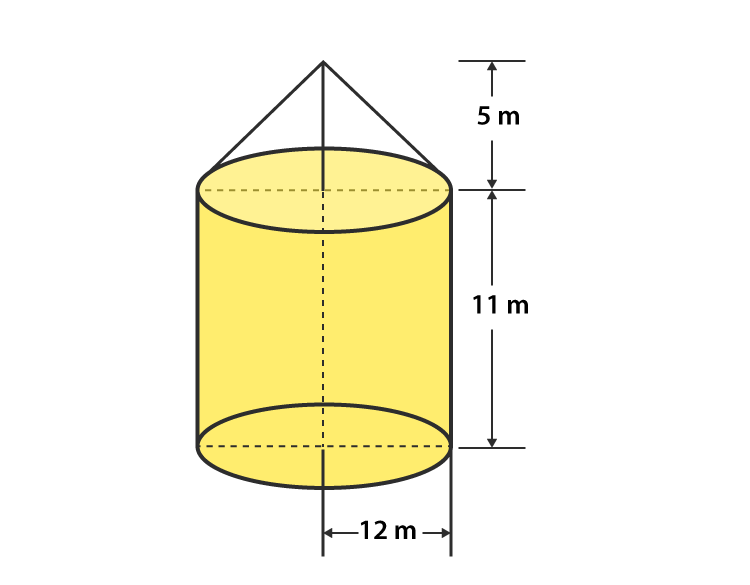Given,

The diameter of the cylinder (also the same for the cone) = 24 m

So, its radius (R) = 24/2 = 12 m

The height of the cylindrical part (H1) = 11m

So, height of the cone part (H2) = 16 – 11 = 5 m

Now,

Vertex of the cone above the ground = 11 + 5 = 16 m

Curved Surface area of the Cone (S1) = πRL = 22/7 × 12 × L

The slant height (L) is given by,

L = √(R2 + H22) = √(122 + 52) = √169

L = 13 m

So,

Curved Surface Area of Cone (S1) = 22/7 × 12 × 13

And,

Curved Surface Area of Cylinder (S2) = 2πRH1

S= 2π(12)(11) m2

Thus, the area of Canvas required for the tent

S = S1 + S= (22/7 × 12 × 13) + (2 × 22/7 × 12 × 11)

S = 490 + 829.38

S = 1319.8 m2

S = 1320 m2

Therefore, the area of canvas required for the tent is 1320 m2

2. A rocket is in the form of a circular cylinder closed at the lower end with a cone of the same radius attached to the top. The cylinder is of radius 2.5 m and height 21 m, and the cone has a slant height 8 m. Calculate the total surface area and the volume of the rocket.

Solution:

Given,

Radius of the cylindrical portion of the rocket (R) = 2.5 m

Height of the cylindrical portion of the rocket (H) = 21 m

Slant Height of the Conical surface of the rocket (L) = 8 m

Curved Surface Area of the Cone (S1) = πRL = π(2.5)(8)= 20π

And,

Curved Surface Area of the Cone (S2) = 2πRH + πR2

S= (2π × 2.5 × 21) + π (2.5)2

S2 = (π × 105) + (π × 6.25)

Thus, the total curved surface area S is

S = S1 + S2

S = (π20) + (π105) + (π6.25)

S = (22/7)(20 + 105 + 6.25) = 22/7 x 131.25

S = 412.5 m2

Therefore, the total Surface Area of the Conical Surface = 412.5 m2

Now, calculating the volume of the rocket,

Volume of the conical part of the rocket (V1) = 1/3 × 22/7 × R2 × h

V1 = 1/3 × 22/7 × (2.5)2 × h

Let h be the height of the conical portion in the rocket.

We know that,

L2 = R+ h2

h2 = L– R2 = 82 – 2.52

h = 7.6 m

Using the value of h, we will get

Volume of the conical part (V1) = 1/3 × 22/7 × 2.52 × 7.6 m2   = 49.67 m2

Next,

Volume of the Cylindrical Portion (V2) = πR2h

V2 = 22/7 × 2.52 × 21 = 412.5 m2

Thus, the total volume of the rocket = V1 + V2

V = 412.5 + 49.67 = 462.17 m2

Hence, the total volume of the Rocket is 462.17 m

3. A tent of height 77 dm is in the form of a right circular cylinder of diameter 36 m and height 44 dm surmounted by a right circular cone. Find the cost of the canvas at Rs. 3.50 per m2

Solution:

Given,

Height of the tent = 77 dm

Height of a surmounted cone = 44 dm

Height of the Cylindrical Portion = Height of the tent – Height of the surmounted Cone

= 77 – 44

= 33 dm = 3.3 m

And, given the diameter of the cylinder (d) = 36 m

So, its radius (r) of the cylinder = 36/2 = 18 m

Let’s consider L as the slant height of the cone.

Then, we know that

L2 = r2 + h2

L2 = 182 + 3.32

L= 324 + 10.89

L2 = 334.89

L = 18.3 m

Thus, the slant height of the cone (L) = 18.3 m

Now, the Curved Surface area of the Cylinder (S1) = 2πrh

S1 = 2π (184.4) m

And, the Curved Surface area of the cone (S2) = πrL

S= π × 18 × 18.3 m

So, the total curved surface of the tent (S) = S+ S2

S = S1 + S2

S = (2π18 × 4.4) + (π18 × 18.3)

S = 1533.08 m2

Hence, the total Curved Surface Area (S) = 1533.08 m2

Next,

The cost of 1 m2 canvas = Rs 3.50

So, 1533.08 m2 of canvas will cost = Rs (3.50 x 1533.08)

= Rs 5365.8

4. A toy is in the form of a cone surmounted on a hemisphere. The diameter of the base and the height of the cone are 6 cm and 4 cm, respectively. Determine the surface area of the toy.

Solution: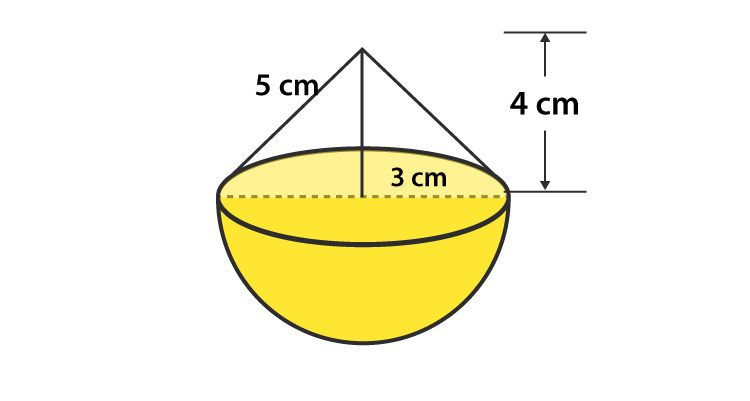Given that,

The height of the cone (h) = 4 cm

Diameter of the cone (d) = 6 cm

So, its radius (r) = 3

Let ‘l’ be the slant height of the cone.

Then, we know that

l2 = r2 + h2

l2 = 32 + 42 = 9 + 16 = 25

l = 5 cm

Hence, the slant height of the cone (l) = 5 cm

So, the curved surface area of the cone (S1) = πrl

S1 = π(3)(5)

S1 = 47.1 cm2

And, the curved surface area of the hemisphere (S2) = 2πr2

S2 = 2π(3)2

S2 = 56.23 cm2

So, the total surface area (S) = S+ S2

S = 47.1 + 56.23

S = 103.62 cm2

Therefore, the curved surface area of the toy is 103.62 cm2

5. A solid is in the form of a right circular cylinder, with a hemisphere at one end and a cone at the other end. The radius of the common base is 3.5 cm, and the height of the cylindrical and conical portions are 10 cm and 6 cm, respectively. Find the total surface area of the solid. (Use π = 22/7)

Solution: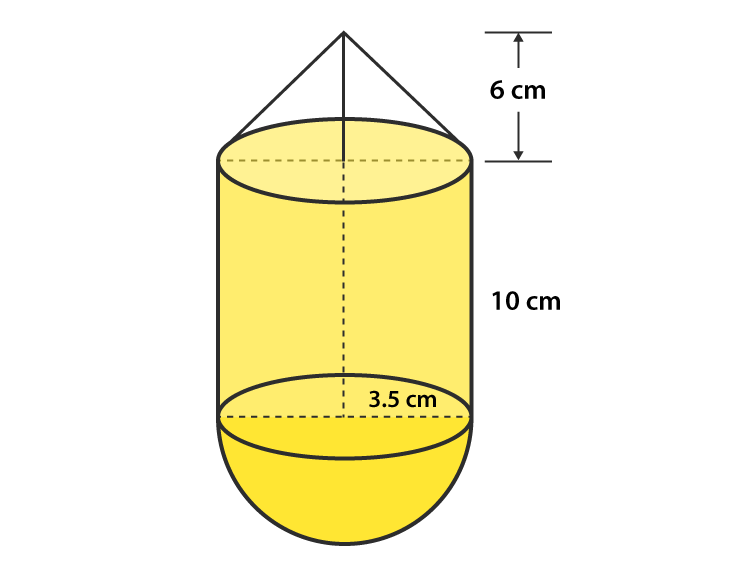Given,

Radius of the common base (r) = 3.5 cm

Height of the cylindrical part (h) = 10 cm

Height of the conical part (H) = 6 cm

Let ‘l’ be the slant height of the cone.

Then, we know that

l2 = r2 + H2

l2 = 3.52 + 62 = 12.25 + 36 = 48.25

l = 6.95 cm

So, the curved surface area of the cone (S1) = πrl

S1 = π(3.5)(6.95)

S1 = 76.38 cm

And, the curved surface area of the hemisphere (S2) = 2πr2

S2 = 2π(3.5)2

S2 = 77 cm2

Next, the curved surface area of the cylinder (S3) = 2πrh

S2 = 2π(3.5) (10)

S2 = 220 cm2

Thus, the total surface area (S) = S+ S2 + S3

S = 76. 38 + 77 + 220 = 373.38 cm2

Therefore, the total surface area of the solid is 373.38 cm2

6. A toy is in the shape of a right circular cylinder with a hemisphere on one end and a cone on the other. The radius and height of the cylindrical parts are 5cm and 13 cm, respectively. The radii of the hemispherical and conical parts are the same as that of the cylindrical part. Find the surface area of the toy if the total height of the toy is 30 cm.

Solution:

Given,

Height of the Cylindrical portion (H) = 13 cm

Radius of the Cylindrical portion (r) = 5 cm

Height of the whole solid = 30 cm

Then,

The curved surface area of the cylinder (S1) = 2πrh

S= 2π(5)(13)

S= 408.2 cm2

Let ‘L’ be the slant height of the cone.

And, the curved surface area of the cone (S2) = πrL

S2 = π(6)L

For the conical part, we have

h = 30 – 13 – 5 = 12 cm

Then, we know that

L2 = r2 + h2

L2 = 52 + 122

L= 25 + 144

L2 = 169

L = 13 m

So,

S2 = π(5)(13) cm2

S2 = 204.28 cm2

Now, the curved surface area of the hemisphere (S3) = 2πr2

S3 = 2π(5)2

S3 = 157.14 cm2

Thus, the total curved surface area (S) = S+ S+ S3

S = (408.2 + 204.28 + 157.14)

S = 769.62 cm2

Therefore, the surface area of the toy is 770 cm2

7. Consider a cylindrical tub having a radius of 5 cm and a length of 9.8 cm. It is full of water. A solid in the form of a right circular cone mounted on a hemisphere is immersed in a tub. If the radius of the hemisphere is 3.5 cm and the height of the cone outside the hemisphere is 5 cm, find the volume of water left in the tub.

Solution:

Given,

The radius of the cylindrical tub (r) = 5 cm

Height of the cylindrical tub (H) = 9.8 cm

Height of the cone outside the hemisphere (h) = 5 cm

Radius of the hemisphere = 3.5 cm

Now, we know that

The volume of the cylindrical tub (V1) = πr2H

V1 = π(5)9.8

V1 = 770 cm3

And, the volume of the hemisphere (V2) = 2/3 × π × r3

V= 2/3 × 22/7 × 3.53

V2 = 89.79 cm3

And, the volume of the hemisphere (V3) = 23 × π × r × 2h

V= 2/3 × 22/7 × 3.52 × 5

V= 64.14 cm3

Thus, total volume (V) = Volume of the cone + Volume of the hemisphere

= V+ V3

V = 89.79 + 64.14 cm

= 154 cm3

So, the total volume of the solid = 154 cm3

In order to find the volume of the water left in the tube, we have to subtract the volume of the hemisphere and the cone from the volume of the cylinder.

Hence, the volume of water left in the tube = V– V2

= 770 – 154

= 616 cm3

Therefore, the volume of water left in the tube is 616 cm3.

8. A circus tent has a cylindrical shape surmounted by a conical roof. The radius of the cylindrical base is 20 cm. The heights of the cylindrical and conical portions are 4.2 cm and 2.1 cm, respectively.  Find the volume of that tent.

Solution: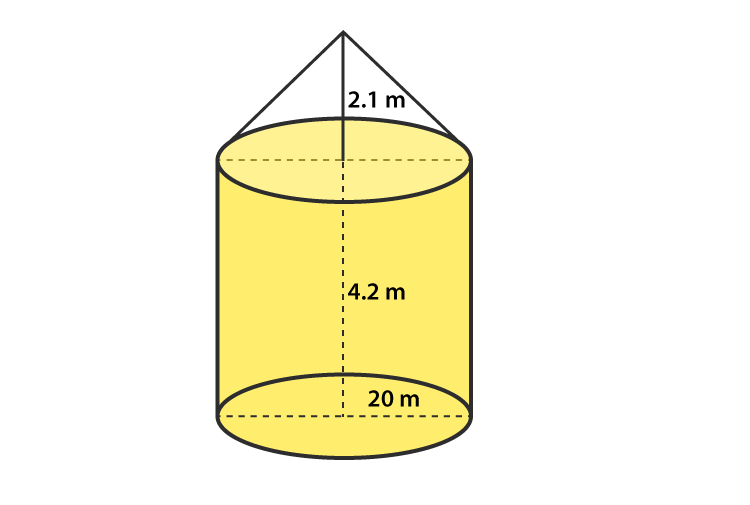Given,

Radius of the cylindrical portion (R) = 20 m

Height of the cylindrical portion (h1) = 4.2 m

Height of the conical portion (h2) = 2.1 m

Now, we know that

Volume of the Cylindrical portion (V1) = πrh1

V1 = π(20)24.2

V= 5280 m3

And, the volume of the conical part (V2) = 1/3 × 22/7 × r2 × h2

V= 13 × 22/7 × 202 × 2.1

V= 880 m3

Thus, the total volume of the tent (V) = volume of the conical portion + volume of the Cylindrical portion

V = V+ V2

V = 6160 m3

Therefore, the volume of the tent is 6160 m3

9. A petrol tank is a cylinder of base diameter 21 cm and length 18 cm, fitted with conical ends, each of axis length 9 cm. Determine the capacity of the tank.

Solution: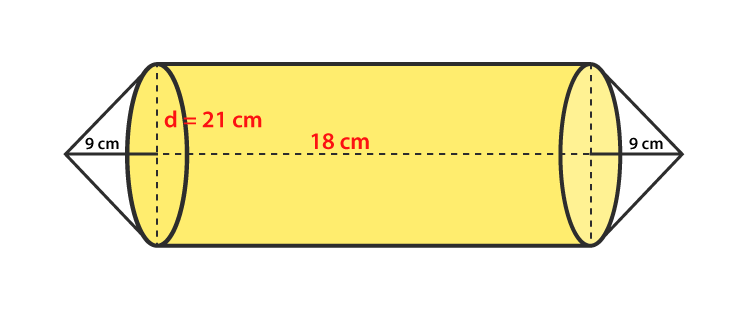Given,

Base diameter of the cylindrical base of the petrol tank = 21 cm

So, its radius (r) = diameter/2 = 21/2 = 10.5 cm

Height of the cylindrical portion of the tank (h1) = 18 cm

Height of the conical portion of the tank (h2) = 9 cm

Now, we know that

The volume of the cylindrical portion (V1) = πrh1

V1 = π(10.5)18

V1 = 6237 cm3

The volume of the conical portion (V2) = 1/3 × 22/7 × r2 × h2

V= 1/3 × 22/7 × 10.52 × 9

V= 1039.5 cm3

Therefore, the total volume of the tank (V) = 2 x volume of a conical portion + volume of the Cylindrical portion

V = V+ V2 = 2 x 1039.5 + 6237

V = 8316 cm3

So, the capacity of the tank = V = 8316 cm

10. A conical hole is drilled in a circular cylinder of height 12 cm and base radius 5 cm. The height and base radius of the cone are also the same. Find the whole surface and volume of the remaining Cylinder.

Solution: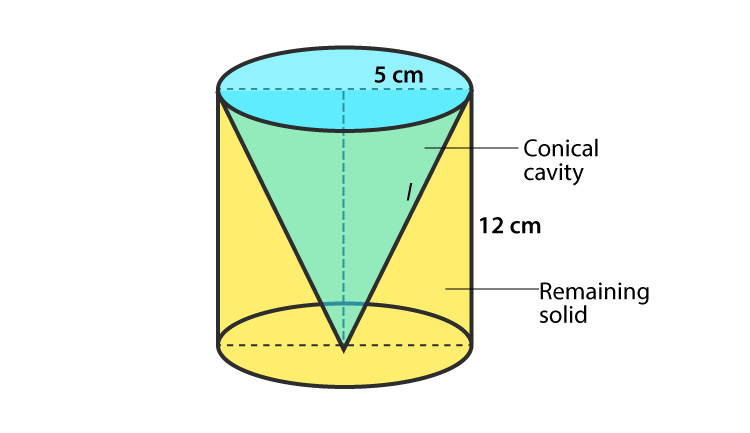Given,

Height of the circular Cylinder (h1) = 12 cm

Base radius of the circular Cylinder (r) = 5 cm

Height of the conical hole = Height of the circular cylinder, i.e., h= h= 12 cm

Base radius of the conical hole = Base radius of the circular Cylinder = 5 cm

Let’s consider L as the slant height of the conical hole.

Then, we know that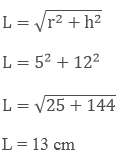Now,

The total surface area of the remaining portion in the circular cylinder (V1) = πr+ 2πrh + πrl

V= π(5) + 2π(5)(12) + π(5)(13)

V= 210 π cm2

And, the volume of the remaining portion of the circular cylinder = Volume of the cylinder – Volume of the conical hole

V = πr2h – 1/3 × 22/7 × r2 × h

V = π(5)2(12) – 1/3 × 22/7 × 52 × 12

V = 200 π cm2

11. A tent is in the form of a cylinder of diameter 20 m and height 2.5 m, surmounted by a cone of equal base and height 7.5 m. Find the capacity of the tent and the cost of the canvas at Rs 100 per square meter.

Solution: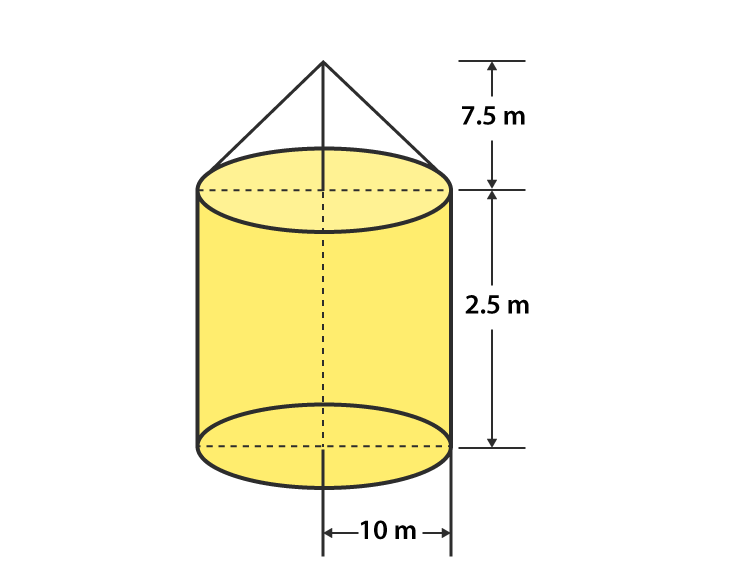Given,

Diameter of the cylinder = 20 m

So, the radius of the cylinder (R) = 10 m

Height of the cylinder (h1) = 2.5 m

Radius of the cone = Radius of the cylinder (r) = 15 m

Height of the Cone (h2) = 7.5 m

Let us consider L as the slant height of the Cone, then, we know that

L2 = r2 + h22

L2 = 152 + 7.52

L= 225 + 56.25

L2 = 281.25

L = 12.5 m

Now,

Volume of the cylinder = πR2h= V1

V= π(10)2.5

V= 250 πm3

Volume of the Cone = 1/3 × 22/7 × r2 × h22 = V2

V= 1/3 × 22/7 × 102 × 7.5

V= 250π m3

So, the total capacity of the tent = Volume of the cylinder + Volume of the cone = V+ V2

V = 250 π + 250 π

V = 500π m3

Hence, the total capacity of the tent is 500π m3

And the total area of canvas required for the tent is S = 2 πRh1 + πrL

S = 2(π)(10)(2.5) + π(10)(12.5)

S = 550 m2

Therefore, the total cost for canvas is (100) (550) = Rs. 55000

12. A boiler is in the form of a cylinder 2 m long with hemispherical ends each of 2 m diameter. Find the volume of the boiler.

Solution: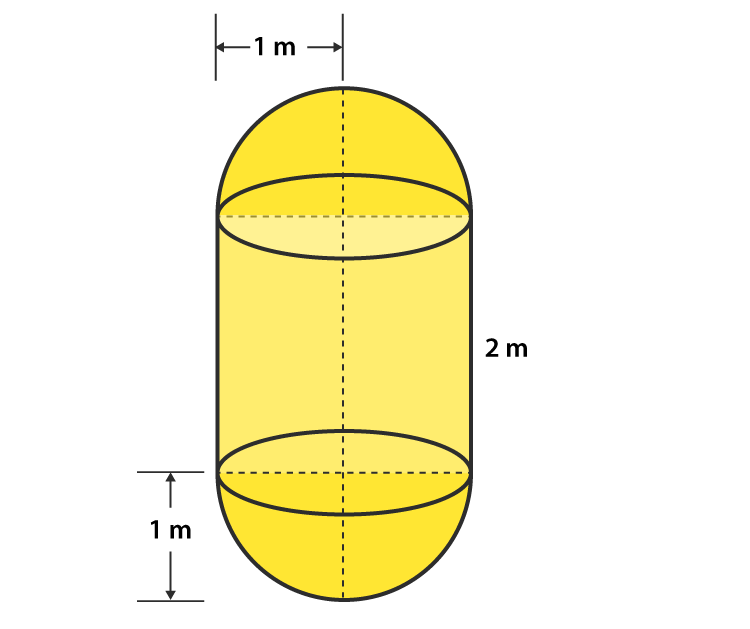Given,

Diameter of the hemisphere = 2 m

So, the radius of the hemisphere (r) = 1 m

Height of the cylinder (h1) = 2 m

And, the volume of the cylinder = πr2h= V1

V= π(1)22

V= 22/7 × 2 = 44/7 m3

At each of the ends of the cylinder, hemispheres are attached.

So totally, there are 2 hemispheres.

Then the volume of two hemispheres = 2 × 2/3 × 22/7 × r3 = V2

V= 2 × 2/3 × 22/7 × 13

V= 22/7 × 4/3 = 88/21 m3

Thus,

The volume of the boiler (V) = Volume of the cylindrical portion + Volume of the two hemispheres

V = V+ V2

V = 44/7 + 88/21

V = 220/21 m3

Therefore, the volume of the boiler 220/21 m3

13. A vessel is a hollow cylinder fitted with a hemispherical bottom of the same base. The depth of the cylinder is 14/3, and the diameter of the hemisphere is 3.5 m. Calculate the volume and the internal surface area of the solid.

Solution:

Given,

Diameter of the hemisphere = 3.5 m

So, the radius of the hemisphere (r) = 1.75 m

Height of the cylinder (h) = 14/3 m

We know that the volume of the cylinder = πr2 h= V1

V= π(1.75)2 x 14/3 m3

The volume of the hemispherical bottom = 2 × 2/3 × 22/7 × r3 = V2

V= 2/3 × 22/7 × 1.753 m3

Therefore,

The total volume of the vessel (V) = Volume of the cylinder + Volume of the hemisphere

V = V+ V2

V = π(1.75)2 x 14/3 + 2/3 × 22/7 × 1.753

V = π(1.75)2 (14/3 + 2/3 x 1.75)

V = 56.15 m2

Hence, the volume of the vessel = V = 56.15 m3

Now,

Internal surface area of solid (S) = Surface area of the cylinder + Surface area of the hemisphere

S = 2 πr h+ 2 πr2

S = 2 π(1.75)(143) + 2 π(1.75)2

S = 70.51 m3

Therefore, the internal surface area of the solid is 70.51 m3

14. A solid is composed of a cylinder with hemispherical ends. If the complete length of the solid is 104 cm and the radius of each of the hemispherical ends is 7 cm, find the cost of polishing its surface at the rate of Rs.10 per dm2.

Solution:

Given,

Radius of the hemispherical end (r) = 7 cm

Height of the solid = (h + 2r) = 104 cm

⇒ h + 2r = 104

⇒ h = 104 − (2 × 7)

So, h = 90 cm

We know that,

The curved surface area of the cylinder (S1) = 2 πr h

S1= 2 π(7)(90)

S1 = 3960 cm2

Next,

Curved surface area of the two hemispheres (S2) = 2 (2πr2)

S2 = 2 x 2π(7)2

S2 = 616 cm2

Therefore,

The total curved surface area of the solid (S) = Curved surface area of the cylinder + Curved surface area of the two hemispheres

S = S1 + S2

S = 3960 + 616

S = 4576 cm= 45.76 dm2

Given that the cost of polishing the 1 dm2 surface of the solid is Rs. 10

So, the cost of polishing the 45.76 dmsurface of the solid = Rs (10 x 45.76) = Rs. 457.6

Therefore,

The cost of polishing the whole surface of the solid is Rs. 457.60

15. A cylindrical vessel of diameter 14 cm and height 42 cm is fixed symmetrically inside a similar vessel of diameter 16cm and height of 42 cm. The total space between the two vessels is filled with cork dust for heat insulation purposes. How many cubic cms of cork dust will be required?

Solution:

Given,

Depth of the cylindrical vessel = Height of the cylindrical vessel = h = 42 cm (common for both)

Inner diameter of the cylindrical vessel = 14 cm

So, the inner radius of the cylindrical vessel = r= 14/2 = 7 cm

Outer diameter of the cylindrical vessel = 16 cm

So, the outer radius of the cylindrical vessel = r= 16/2 = 8 cm

Now,

The volume of the cylindrical vessel

V = π(r22 – r12 ) x h

= π(82 – 72 ) x 42

= 22/7 x 15 x 42

V = 1980 cm3

Therefore, the total space between the two vessels is 1980 cm3, which is also the amount of cork dust required.

16. A cylindrical road roller made of iron is 1 m long. Its internal diameter is 54 cm, and the thickness of the iron sheet used in making the roller is 9 cm. Find the mass of the road roller, if 1 cm3 of the iron has 7.8 gm mass.

Solution:

Given,

Height/length of the cylindrical road roller = h = 1 m = 100 cm

Internal Diameter of the cylindrical road roller = 54 cm

So, the internal radius of the cylindrical road roller = 27 cm = r

Also given, the thickness of the road roller (T) = 9 cm

Let us assume that the outer radii of the cylindrical road roller be R.

T = R – r

9 = R – 27

R = 27 + 9

R = 36 cm

Now,

The volume of the iron sheet (V) = π × (R2 − r2) × h

V = π × (362 − 272) × 100

V = 1780.38 cm3

Hence, the volume of the iron sheet = 1780.38 cm3

It’s given that the mass of 1 cmof the iron sheet = 7.8 gm

So, the mass of 1780.38 cm3 of the iron sheet = 1388696.4gm = 1388.7 kg

Therefore, the mass of the road roller is 1388.7 kg

17. A vessel in the form of a hollow hemisphere mounted by a hollow cylinder. The diameter of the hemisphere is 14 cm, and the total height of the vessel is 13cm. Find the inner surface area of the vessel.

Solution:

Given,

Diameter of the hemisphere = 14 cm

So, the radius of the hemisphere = 7 cm

Total height of the vessel = 13 cm = h + r

Now,

Inner surface area of the vessel = 2 π r (h + r)

= 2 (22/7)(7) (13)

= 572 cm

Therefore, the inner surface area of the vessel is 572 cm2

18. A toy is in the form of a cone of radius 3.5 cm mounted on a hemisphere of the same radius. The total height of the toy is 15.5 cm. Find the total surface area of the toy.

Solution:

Given,

Radius of the conical portion of the toy = 3.5 cm = r

Total height of the toy = 15.5 cm = H

If H is the length of the conical portion,

Then,

Length of the cone (h) = H – r = 15.5 – 3.5 = 12 cm

Now, we know that

The curved surface area of the cone (S1) = πrL, where L is the slant height of the cone.

L2 = r2 + h2

L2 = 3.52 + 122

L2 = 12.25 + 144 = 156.25

L = 12.5

So,

S1 = π (3.5)(12.5)

S1 = 137.5 cm2

Next, the curved surface area of the hemisphere (S2) = 2πr2

S2 = 2π (3.5)2

S2 = 77 cm2

Therefore,

The total surface area of the toy (S) = Curved surface area of the cone + curved surface area of the hemisphere

S = S1 + S2

S = 137.5 + 77

S = 214.5 cm2

Hence, the total surface area of the children’s toy is 214.5 cm

### RD Sharma Solutions for Class 10 Maths Chapter 16 Exercise 16.3 Page No: 16.60

1. A bucket has top and bottom diameters of 40 cm and 20 cm, respectively. Find the volume of the bucket if its depth is 12 cm. Also, find the cost of the tin sheet used for making the bucket at the rate of Rs 1.20 per dm2.

Solution:

Given,

Diameter to top of bucket = 40 cm

So, the radius (r1) = 40/2 = 20 cm

Diameter of the bottom part of the bucket = 20 cm

So, the radius (r2) = 30/2 = 10cm

Depth of the bucket (h) = 12 cm

Volume of the bucket = 1/3 π(r22 + r12 + r1 r)h

= π/3(202 + 102 + 20 × 10)12

= 8800 cm3

Now,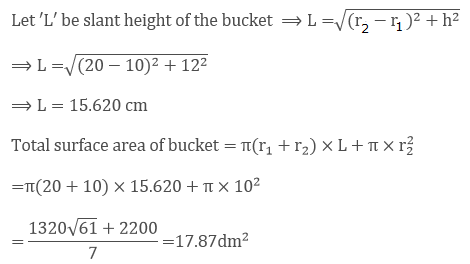Given that the cost of the tin sheet used for making bucket per dm2 = Rs 1.20

So, the total cost for 17.87dm2 = 1.20 × 17.87 = Rs 21.40

Therefore, the cost of the tin sheet used for making the bucket is Rs 21.40

2. A frustum of a right circular cone has a diameter of base 20 cm, of top 12 cm and height 3 cm. Find the area of its whole surface and volume.

Solution:

Given,

Base diameter of the cone (d1) = 20 cm

So the radius (r1) = 20/2 cm = 10 cm

Top diameter of THE Cone (d2) = 12 cm

So, the radius (r2) = 12/2 cm = 6 cm

Height of the cone (h) = 3 cm

Volume of the frustum of a right circular cone = 1/3 π(r22 + r12 + r1 r)h

= π/3(102 + 6+ 10 × 6)3

= 616 cm3

Let ‘L’ be the slant height of the cone, then we know that

L = √(r1 – r21)2 + h2

L = √(10 – 6)2 + 32

L = √(16 + 9)

L = 5cm

So, the slant height of cone (L) = 5 cm

Thus,

Total surface area of the frustum = π(r1 + r2) x L + π r12 + π r22

= π(10 + 6) × 5 + π × 102 + π × 62

= π(80 + 100 + 36)

= π(216)

= 678.85 cm2

3. The slant height of the frustum of a cone is 4 cm, and the perimeters of its circular ends are 18 cm and 6 cm. Find the curved surface of the frustum.

Solution:

Given,

The slant height of the frustum of THE cone (l) = 4 cm

Let the ratio of the top and bottom circles be r1 and r2

And given the perimeters of its circular ends as 18 cm and 6 cm

⟹ 2πr1 = 18 cm; 2πr2 = 6 cm

⟹ πr1= 9 cm and πr2 = 3 cm

We know that,

Curved surface area of frustum of a cone = π(r1 + r2)l

= π(r+ r2)l

= (πr1+πr2)l = (9 + 3) × 4 = (12) × 4 = 48 cm2

Therefore, the curved surface area of the frustum = 48 cm2

4. The perimeters of the ends of a frustum of a right circular cone are 44 cm and 33 cm. If the height of the frustum is 16 cm, find its volume, the slant surface and the total surface.

Solution:

Given,

Perimeter of the upper end = 44 cm

2 π r1 = 44

2(22/7) r1 = 44

r1 = 7 cm

Perimeter of the lower end = 33 cm

2 π r2 = 33

2(22/7) r2 = 33

r2 = 21/4 cm

Now,

Let the slant height of the frustum of a right circular cone is L.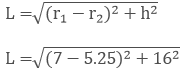L = 16.1 cm

So, the curved surface area of the frustum cone = π(r1 + r2)l

= π(7 + 5.25)16.1

Curved surface area of the frustum cone = 619.65 cm3

Next,

The volume of the frustum cone = 1/3 π(r22 + r12 + r1 r)h

= 1/3 π(72 + 5.252 + (7) (5.25) ) x 16

= 1898.56 cm3

Thus, volume of the cone = 1898.56 cm3

Finally, the total surface area of the frustum cone

= π(r1 + r2) x L + π r12 + π r22

= π(7 + 5.25) × 16.1 + π7+ π5.252

= π(7 + 5.25) × 16.1 + π(72 + 5.252) = 860.27 cm2

Therefore, the total surface area of the frustum cone is 860.27 cm2

5. If the radii of the circular ends of a conical bucket which is 45 cm high and the radii of the 2 circular ends are 28 cm and 7 cm, find the capacity of the bucket.

Solution:

Given,

Height of the conical bucket = 45 cm

Radii of the 2 circular ends of the conical bucket are 28 cm and 7 cm

So, r1 = 28 cm r2 = 7 cm

Volume of the conical bucket = 1/3 π(r12 + r22 + r1 r)h

= 1/3 π(282 + 72 + 28 × 7)45 = 15435π

Therefore, the volume/ capacity of the bucket is 48510 cm3.

6. The height of a cone is 20 cm. A small cone is cut off from the top by a plane parallel to the base. If its volume is 1/125 of the volume of the original cone, determine at what height above the base the section is made.

Solution: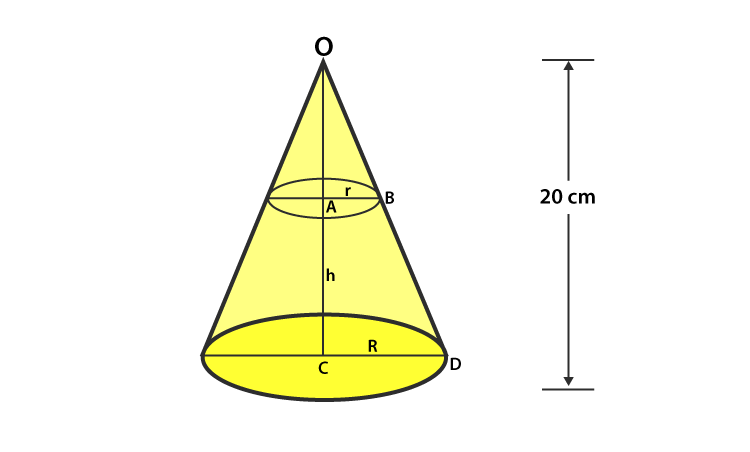Let the radius of the small cone be r cm

Radius if the big cone = R cm

Given, height of the big cone = 20 cm

Let the height of the section made = h cm

Then, the height of the small cone will be = (20 – h) cm

Now,

In △OAB and △OCD

∠AOB = ∠COD [common]

∠OAB = ∠OCD [each 90o]

Then, OAB ~ △OCD [by AA similarity]

So, by C.P.S.T, we have

OA/ OC = AB/ CD

(20 – h)/ 20 = r/ R …… (i)

Also given,

Volume of small cone = 1/125 x Volume of big cone

1/3 π r2(20 – h) = 1/125 x 1/3 πR2 x 20

r2/ R2 = 1/125 x 20/ (20 – h) [From (i)]

(20 – h)2/ 202 = 1/125 x 20/20 – h

(20 – h)3 = 203/ 125

20 – h = 20/5

20 – h = 4

h = 20 – 4 = 16 cm

Therefore, it’s found that the section was made at the height of 16 cm above the base.

7. If the radii of the circular ends of a bucket 24 cm high are 5 and 15 cm, respectively, find the surface area of the bucket.

Solution:

Given,

Height of the bucket (h) = 24 cm

Radius of the circular ends of the bucket is 5 cm and 15 cm

So, r1 = 5 cm; r2 = 15 cm

Let ‘L’ be the slant height of the bucket.

Then, we know that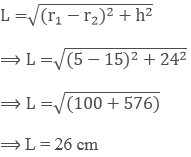Now,

Curved surface area of the bucket

= π(r1 + r2)l + πr12

= π(5 + 15)26 + π52 = π(520 + 25) = 545π cm2

Therefore, the curved surface area of the bucket = 545π cm2

8. The radii of circular bases of a frustum of a right circular cone are 12 cm and 3 cm, and the height is 12 cm. Find the total surface area and volume of the frustum.

Solution:

Given the height of the frustum cone = 12 cm

Radii of a frustum cone are 12 cm and 3 cm

So, r1 = 12 cm; r2 = 3 cm

Let the slant height of the frustum cone be ‘L’.

Then, we know that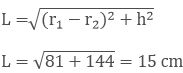L = 15 cm

Now, the total surface area of frustum of a cone = π (r1 + r2) x L + π r12 + π r22

= π (12 + 3)15 + π122 + π32

Thus, total surface area of the frustum = 378 π cm2

Next,

Volume of the frustum cone = 1/3 π(r22 + r12 + r1 r)h

= 1/3 π(122 + 32 + 12 × 3) × 12 = 756π cm3

Therefore, the volume of the frustum cone = 756 π cm3

9. A tent consists of a frustum of a cone capped by a cone. If radii of the ends of the frustum are 13 m and 7 m, the height of the frustum is 8 m, and the slant height of the conical cap is 12 m, find the canvas required for the tent.

Solution:

Given,

Height of frustum (h) = 8 m

Radii of the frustum cone are 13 cm and 7 cm

So, r1 = 13 cm and r2 = 7 cm

Let ‘L’ be the slant height of the frustum cone.

Then, we know that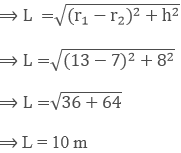Curved surface area of the frustum (s1) = π(r1 + r2) × L =  π(13 + 7) × 10 = 200 π m2

Then, given the slant height of the conical cap = 12 m

Base radius of upper cap cone = 7 m

So, the curved surface area of the upper cap cone (s2) = πrl = π × 7 × 12 = 264 m2

Thus, the total canvas required for tent (S) = s1 + s2

S = 200π + 264 = 892.57 m2

Therefore, the canvas required for the tent is 892.57 m2.

10. A milk container of height 16 cm is made of a metal sheet in the form of a frustum of a cone with radii of its lower and upper ends as 8 cm and 20 cm, respectively. Find the cost of milk at the rate of Rs.44 per litre which the container can hold.

Solution:

Given,

A milk container in the form of a frustum of a cone.

The radius of the lower end (r1) = 8 cm

The radius of the upper end (r2) = 20 cm

Let h be its height, h = 16 cm

Then, the capacity of the container = Volume of a frustum of the cone

= 1/3 π(r22 + r12 + r1 r)h

= 1/3 π(202 + 82 + (20) (8) ) x 16

= 10459.42 cm3

= 10.46 litres

Now, given that cost of 1 litre of milk = Rs 44

Then the cost of 10.46 litres of milk = Rs (44 x 10.46) = Rs 460.24

11. A bucket is in the form of a frustum of a cone of height 30 cm with radii of its lower and upper ends as 10 cm and 20 cm, respectively. Find the capacity and surface area of the bucket. Also, find the cost of milk, which can completely fill the container at the rate of Rs.25 per litre.

Solution:

Let R and r be the radii of the top and base of the bucket, respectively,

Let h be its height.

Then, we have R = 20 cm, r = 10 cm, h = 30 cm

Capacity of the bucket = Volume of the frustum of the cone

= 1/3 π(R2 + r2 + R r )h

= 1/3 π(202 + 102 + 20 x 10 ) x 30

= 3.14 x 10 (400 + 100 + 200)

= 21980 cm3 = 21.98 litres

Now,

Surface area of the bucket = CSA of the bucket + Surface area of the bottom

= π l (R + r) + πr2

We know that,

l = √h2 + (R – r)2

= √[302 + (20 – 10)2] = √(900 + 100)

= √1000 = 31.62 cm

So,

The Surface area of the bucket = (3.14) x 31.62 x (20 + 10) + (3.14) x 102

= 2978.60 + 314

= 3292.60 cm2

Next, given that the cost of 1 litre milk = Rs 25

Thus, the cost of 21.98 litres of milk = Rs (25 x 21.98) = Rs 549.50

### Key features of RD Sharma Solutions are given below:

• RD Sharma Solutions offer accurate answers to each and every question of the textbook to help students in effective revision.
• Students who want to obtain proficiency in solving problems are suggested to practise RD Sharma Solutions on a regular basis.
• Step-by-step explanations boost skills in solving complex problems effortlessly among students.
• Regular practice of these solutions helps students to understand the concepts in detail and clear their doubts instantly.
• For more conceptual knowledge, students can make use of solutions PDF as per their requirements.

## Frequently Asked Questions on RD Sharma Solutions for Class 10 Maths Chapter 16

### Where can I get the correct RD Sharma Solutions for Class 10 Maths Chapter 16?

Students can find correct and accurate RD Sharma Solutions for Class 10 Maths Chapter 16 on BYJU’S website. RD Sharma textbooks are the best reference materials which provide students and teachers with a wide range of practice questions to answer. The solutions are prepared with utmost care to help students understand the concepts easily and score well in their board exams.

### Are RD Sharma Solutions for Class 10 Maths Chapter 16 the best source of study material for exam preparation?

Yes, RD Sharma Solutions are the best source of study material for CBSE board exam preparation. The subject experts at BYJU’S present the concepts in a clear and precise manner based on the IQ level of students. Following RD Sharma Solutions on a daily basis helps students to score excellent marks in examinations. Our solution module utilises various practical examples to explain all the exercise questions in simple and easily understandable language.

### Where can I download RD Sharma Solutions for Class 10 Maths Chapter 16?

RD Sharma Solutions for Class 10 Maths Chapter 16 can be viewed both in online and offline modes from the BYJU’S website. A team of expert tutors at BYJU’S have formulated the solutions for all the questions of the textbook. RD Sharma Solutions for Class 10 Maths are formulated in accordance with the latest CBSE guidelines in simple language to help students understand the concepts with ease.